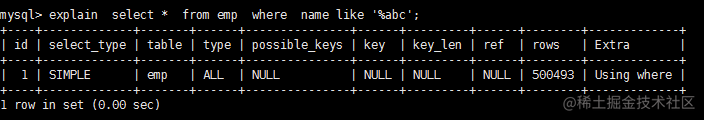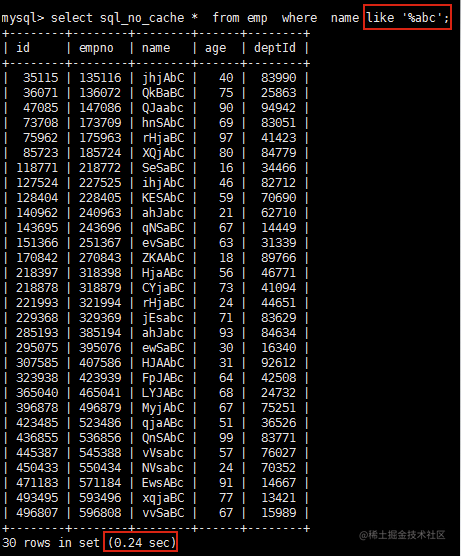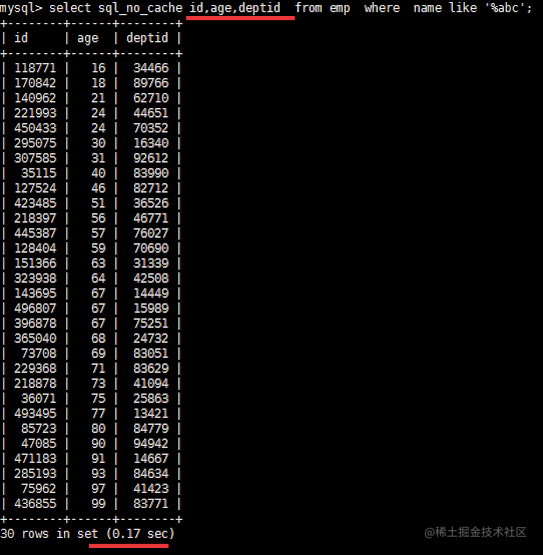# MySQL索引优化

## 环境搭建

### 建表语句

``````
CREATE TABLE `dept` (
`id` INT(11) NOT NULL AUTO_INCREMENT,
`deptName` VARCHAR(30) DEFAULT NULL,
ceo INT NULL ,
PRIMARY KEY (`id`)
) ENGINE=INNODB AUTO_INCREMENT=1 DEFAULT CHARSET=utf8;

CREATE TABLE `emp` (
`id` INT(11) NOT NULL AUTO_INCREMENT,
`empno` INT NOT NULL ,
`name` VARCHAR(20) DEFAULT NULL,
`age` INT(3) DEFAULT NULL,
`deptId` INT(11) DEFAULT NULL,
PRIMARY KEY (`id`)
#CONSTRAINT `fk_dept_id` FOREIGN KEY (`deptId`) REFERENCES `t_dept` (`id`)

### 创建函数

• show variables like 'log_bin_trust_function_creators';
• set global log_bin_trust_function_creators=1;

``````DELIMITER \$\$
CREATE FUNCTION rand_string(n INT) RETURNS VARCHAR(255)
BEGIN
DECLARE chars_str VARCHAR(100) DEFAULT 'abcdefghijklmnopqrstuvwxyzABCDEFJHIJKLMNOPQRSTUVWXYZ';
DECLARE return_str VARCHAR(255) DEFAULT '';
DECLARE i INT DEFAULT 0;
WHILE i < n DO
SET return_str =CONCAT(return_str,SUBSTRING(chars_str,FLOOR(1+RAND()*52),1));
SET i = i + 1;
END WHILE;
RETURN return_str;
END \$\$
#假如要删除
#drop function rand_string;

``````#用于随机产生多少到多少的编号
DELIMITER \$\$
CREATE FUNCTION  rand_num (from_num INT ,to_num INT) RETURNS INT(11)
BEGIN
DECLARE i INT DEFAULT 0;
SET i = FLOOR(from_num +RAND()*(to_num -from_num+1));
RETURN i;
END\$\$
#假如要删除
#drop function rand_num;

SELECT rand_num(50,100);

### 创建调用存储过程

``````DELIMITER \$\$
CREATE PROCEDURE insert_emp(START INT, max_num INT)
BEGIN
DECLARE i INT DEFAULT 0;
#set autocommit =0 把autocommit设置成0
SET autocommit = 0;
REPEAT
SET i = i + 1;
INSERT INTO emp (empno, NAME ,age ,deptid ) VALUES ((START+i) ,rand_string(6), rand_num(30,50),rand_num(1,10000));
UNTIL i = max_num
END REPEAT;
COMMIT;
END\$\$
#删除
# DELIMITER ;
# drop PROCEDURE insert_emp;

#执行存储过程，往emp表添加50万条数据
DELIMITER ;
CALL insert_emp(100000,500000);

``````#执行存储过程，往dept表添加随机数据
DELIMITER \$\$
CREATE PROCEDURE `insert_dept`(max_num INT)
BEGIN
DECLARE i INT DEFAULT 0;
SET autocommit = 0;
REPEAT
SET i = i + 1;
INSERT INTO dept ( deptname,address,ceo ) VALUES (rand_string(8),rand_string(10),rand_num(1,500000));
UNTIL i = max_num
END REPEAT;
COMMIT;
END\$\$
#删除
# DELIMITER ;
# drop PROCEDURE insert_dept;

#执行存储过程，往dept表添加1万条数据
DELIMITER ;
CALL insert_dept(10000);

### 删除索引

``````DELIMITER \$\$
CREATE PROCEDURE `proc_drop_index`(dbname VARCHAR(200),tablename VARCHAR(200))
BEGIN
DECLARE done INT DEFAULT 0;
DECLARE ct INT DEFAULT 0;
DECLARE _index VARCHAR(200) DEFAULT '';
DECLARE _cur CURSOR FOR SELECT index_name FROM information_schema.STATISTICS   WHERE table_schema=dbname AND table_name=tablename AND seq_in_index=1 AND index_name<>'PRIMARY' ;
OPEN _cur;
FETCH _cur INTO _index;
WHILE _index<>'' DO
SET @str = CONCAT("drop index ",_index," on ",tablename );
PREPARE sql_str FROM @str ;
EXECUTE  sql_str;
DEALLOCATE PREPARE sql_str;
SET _index='';
FETCH _cur INTO _index;
END WHILE;
CLOSE _cur;
END\$\$

#CALL proc_drop_index("dbname","tablename");

## 单表使用索引及常见索引失效

### 案例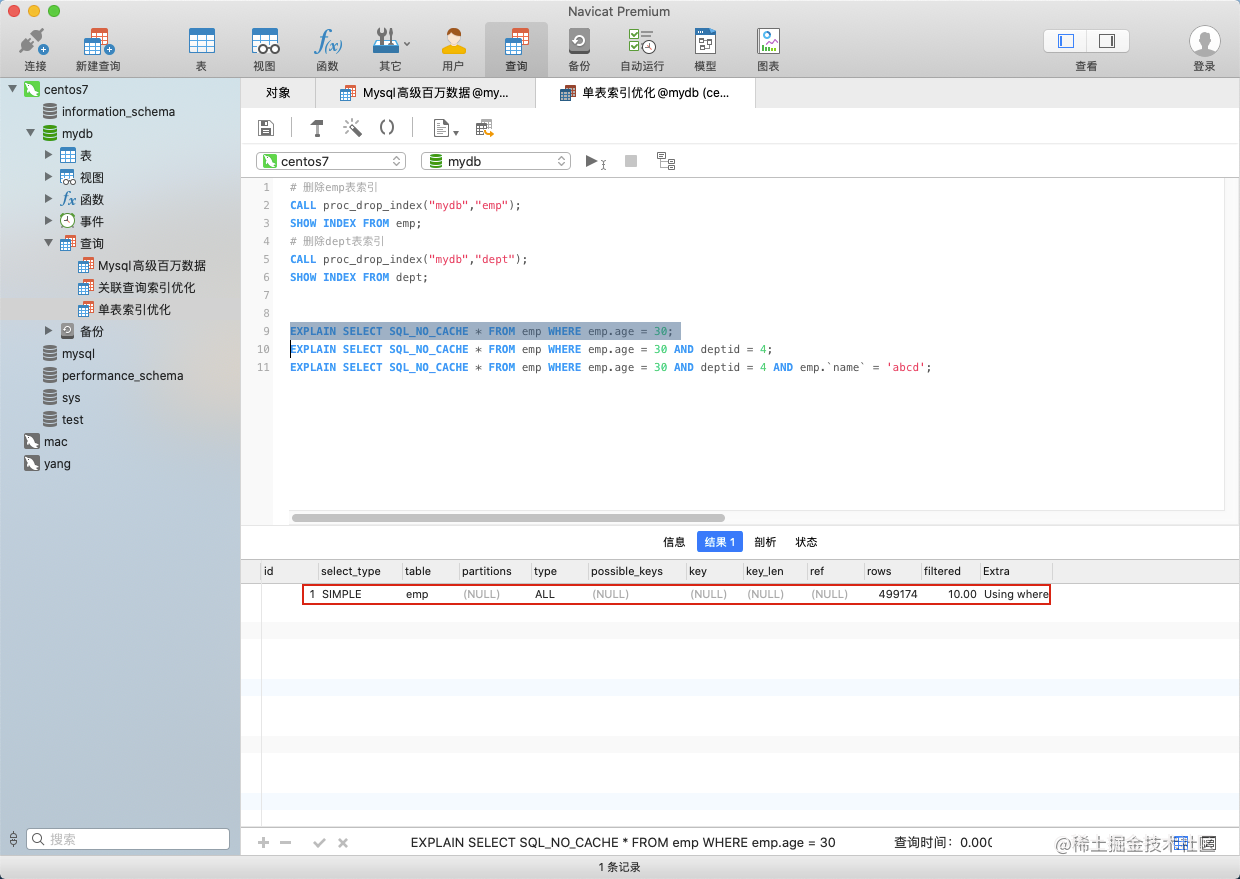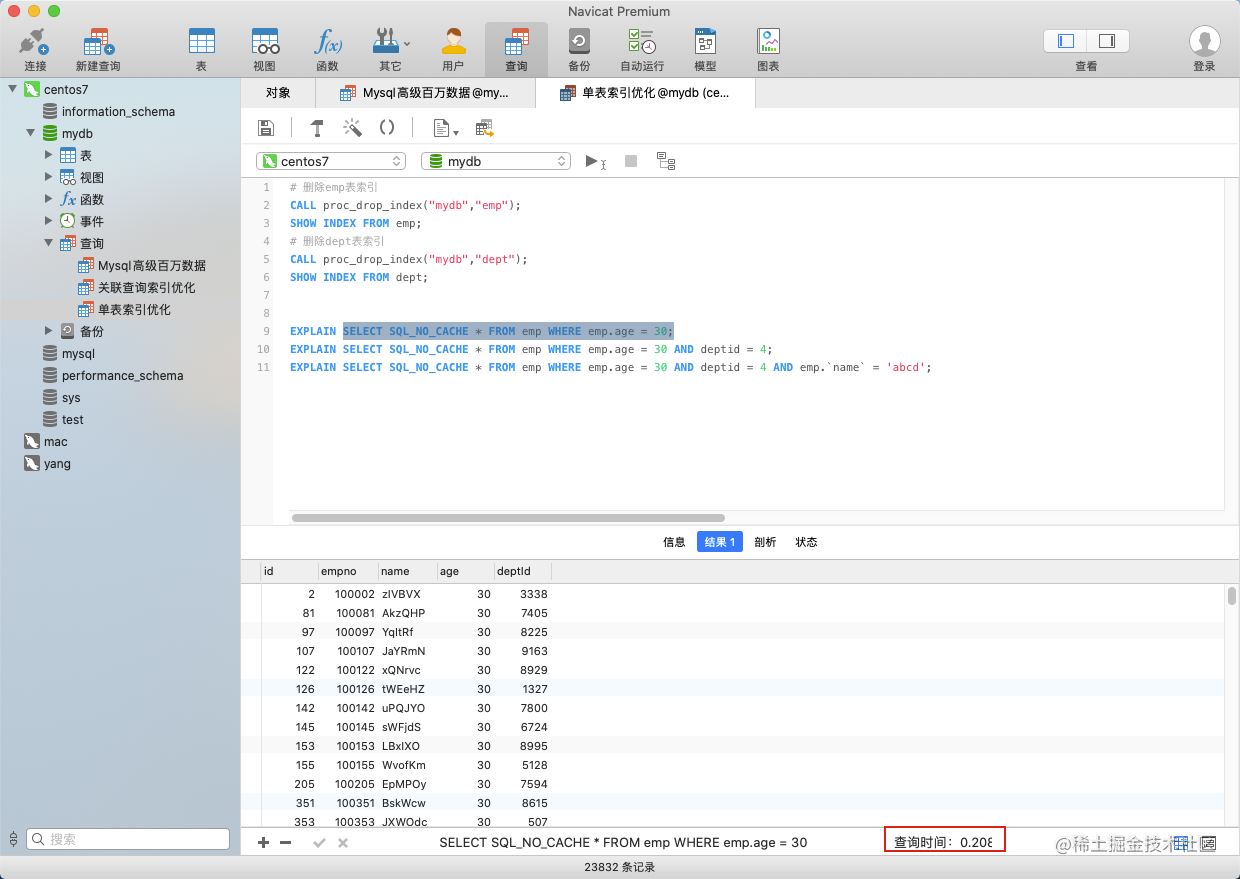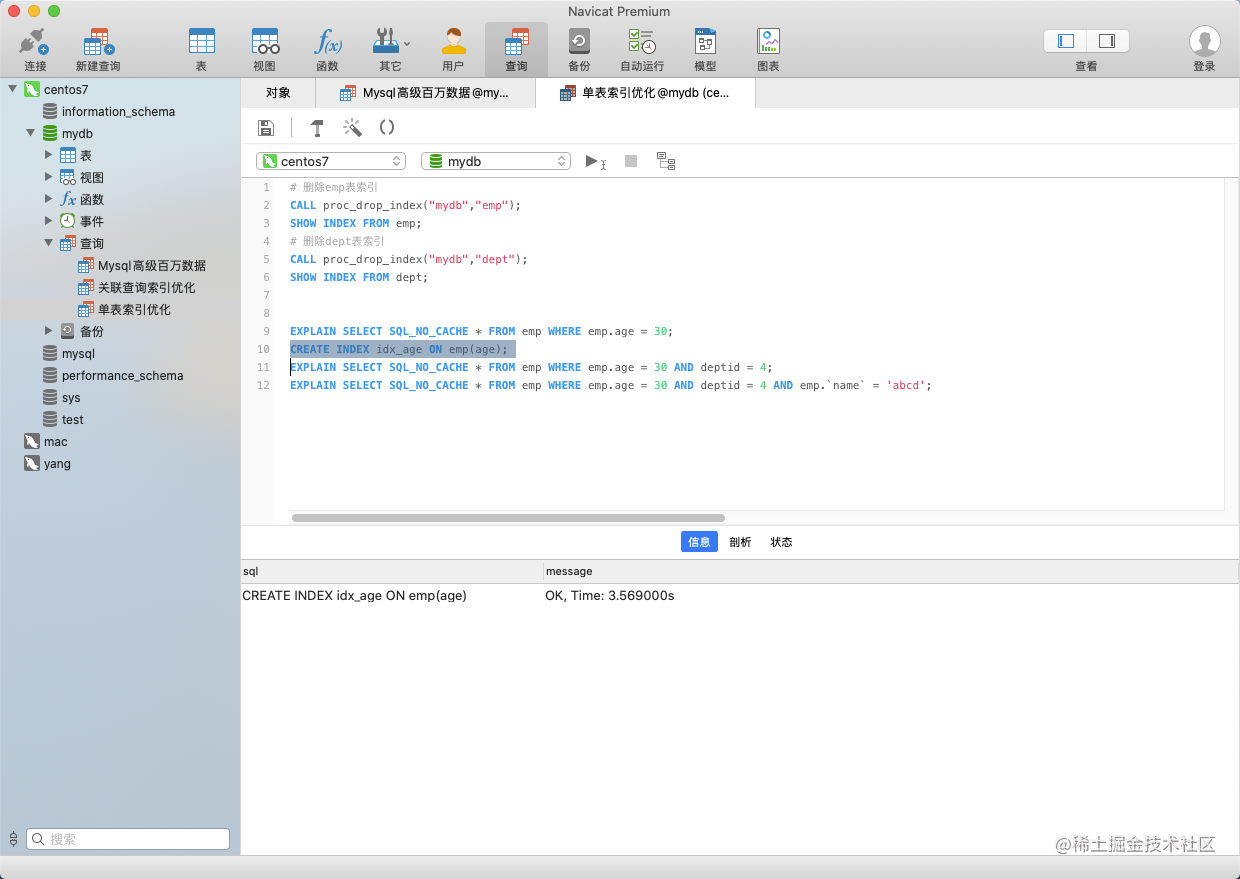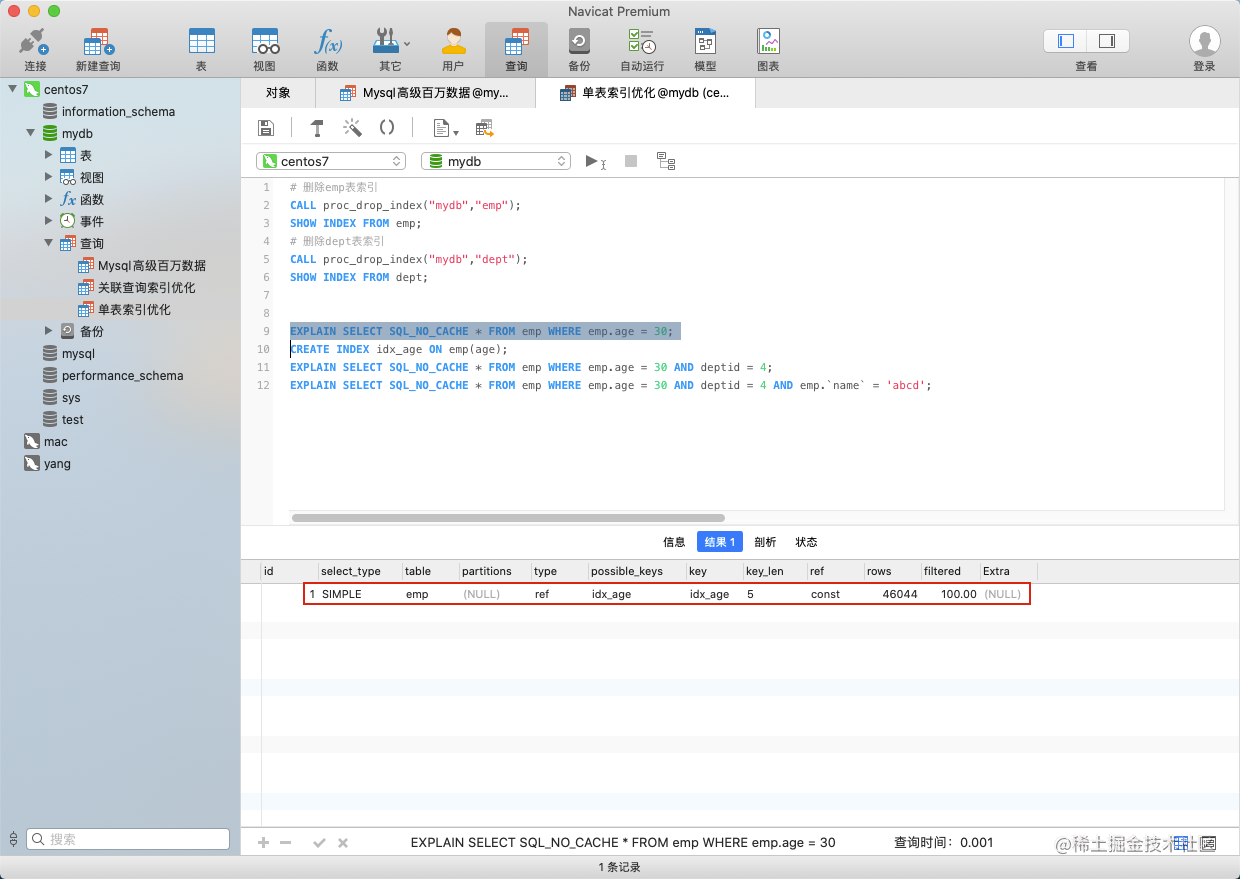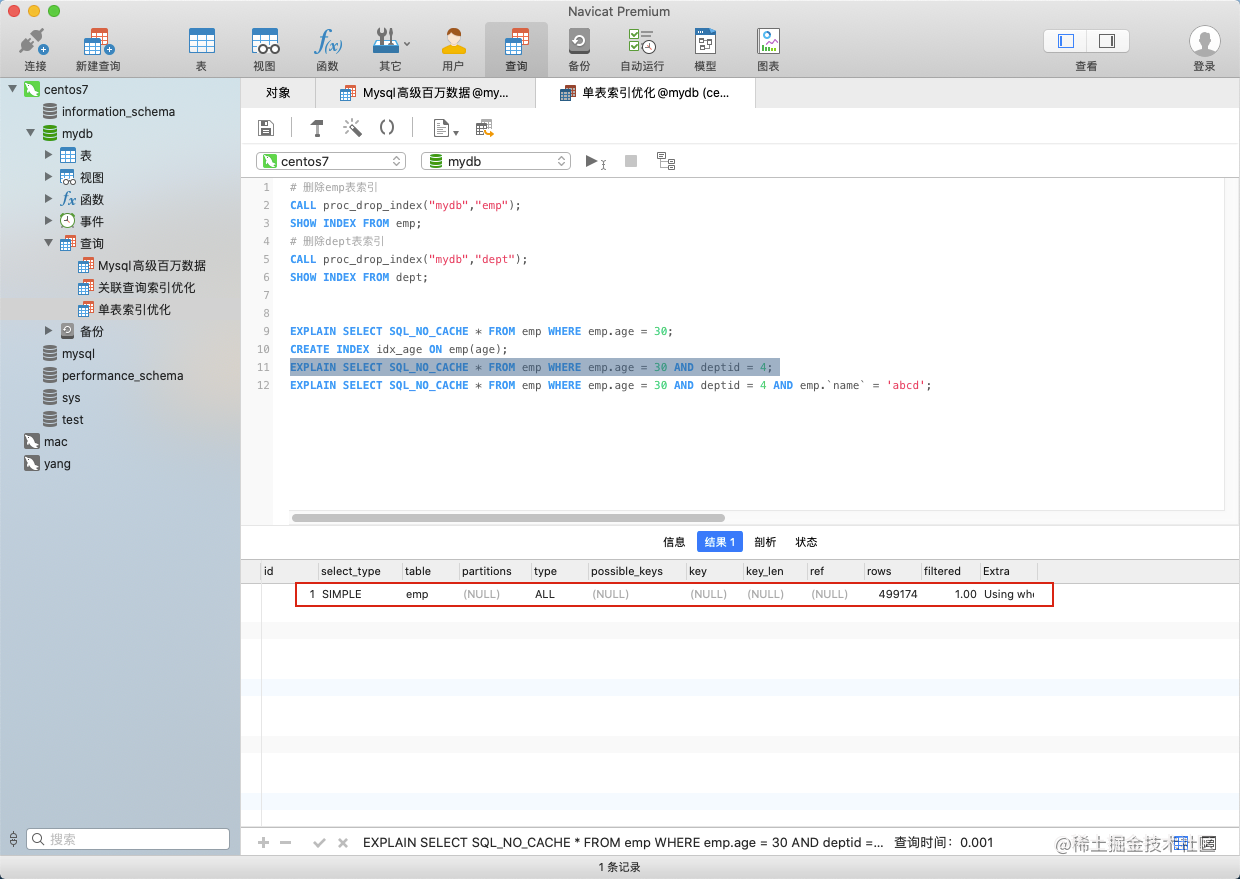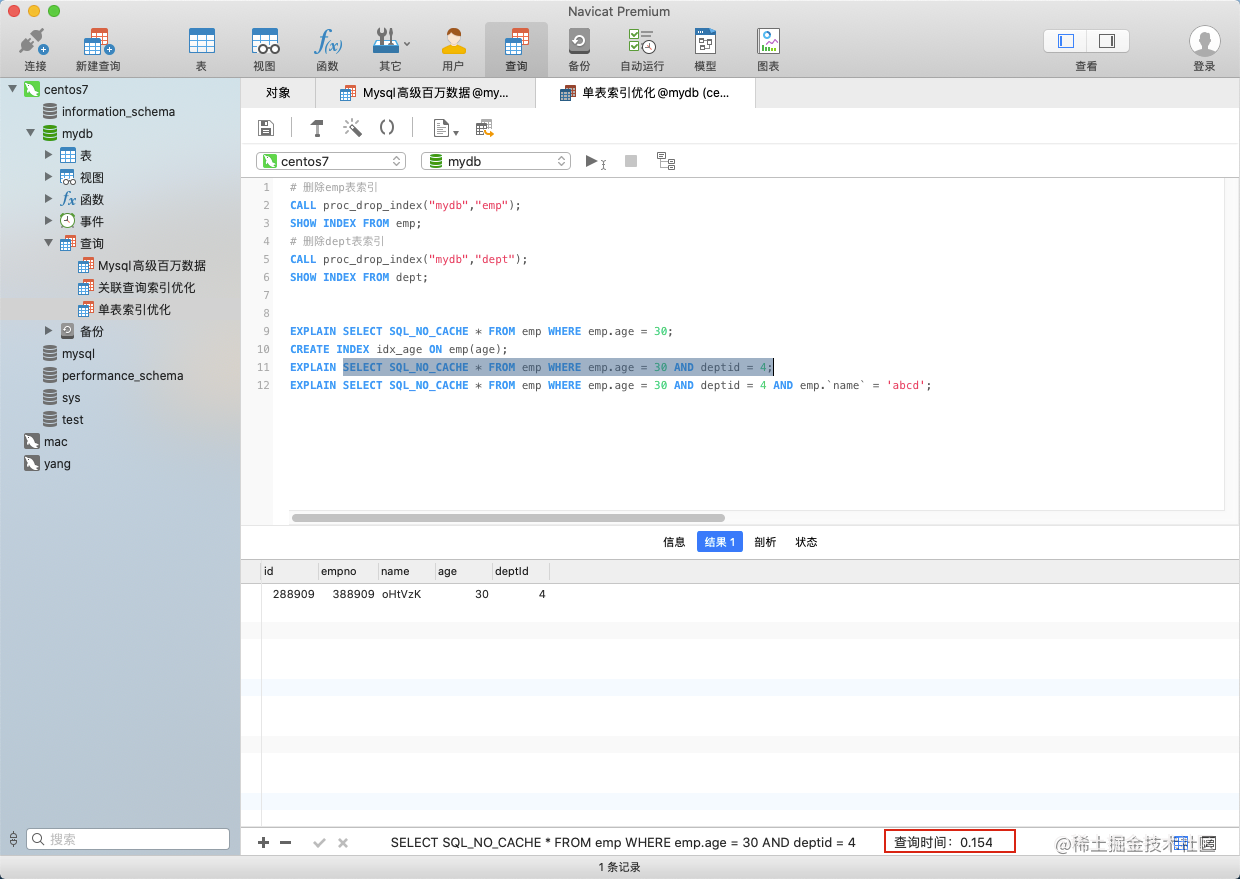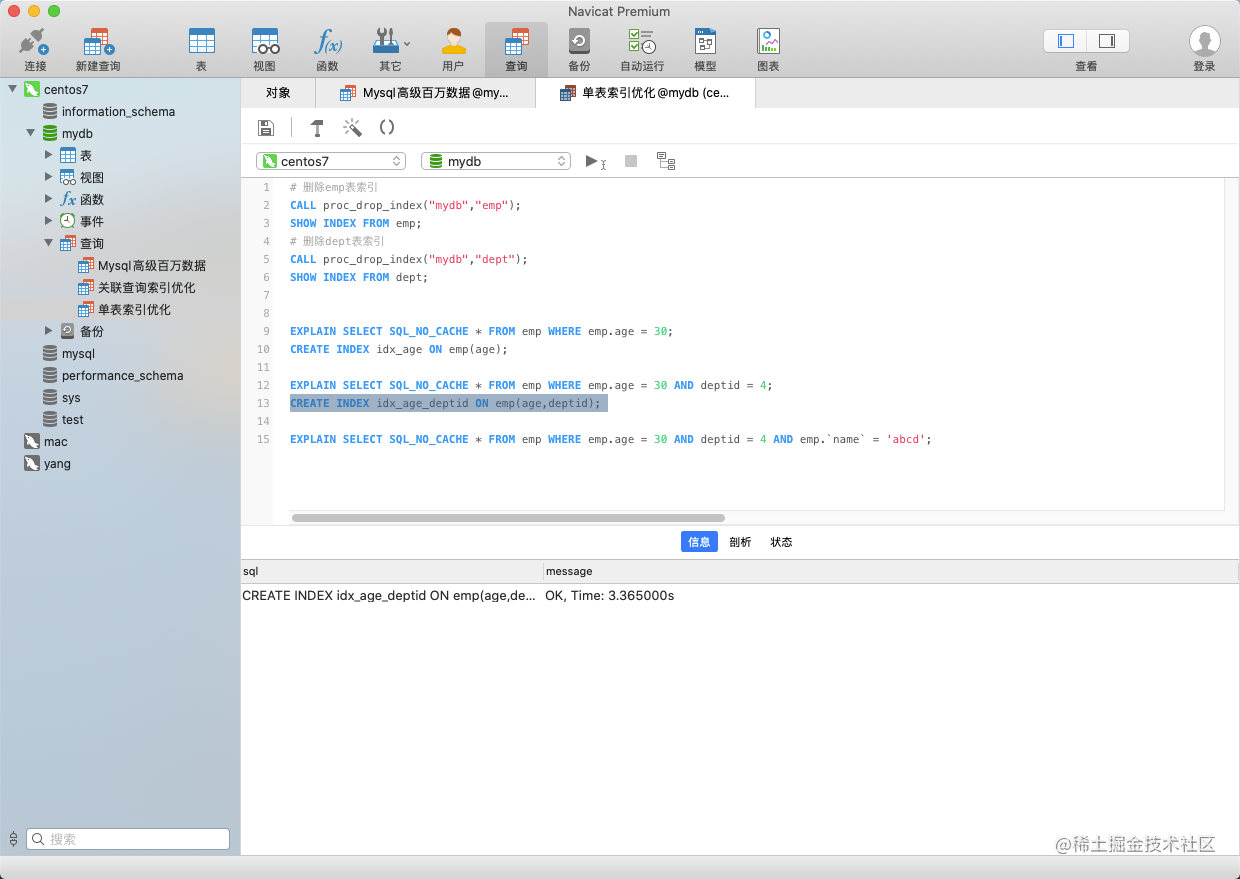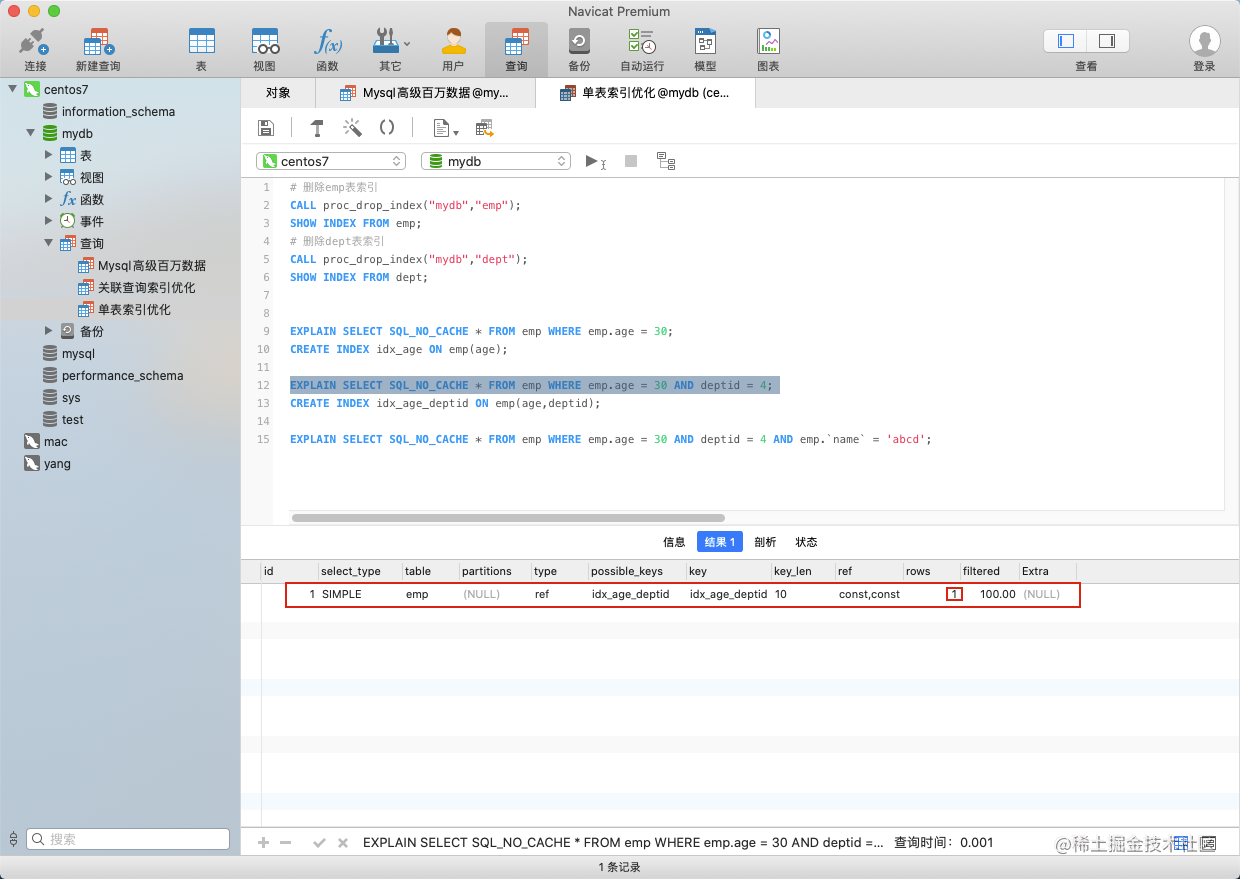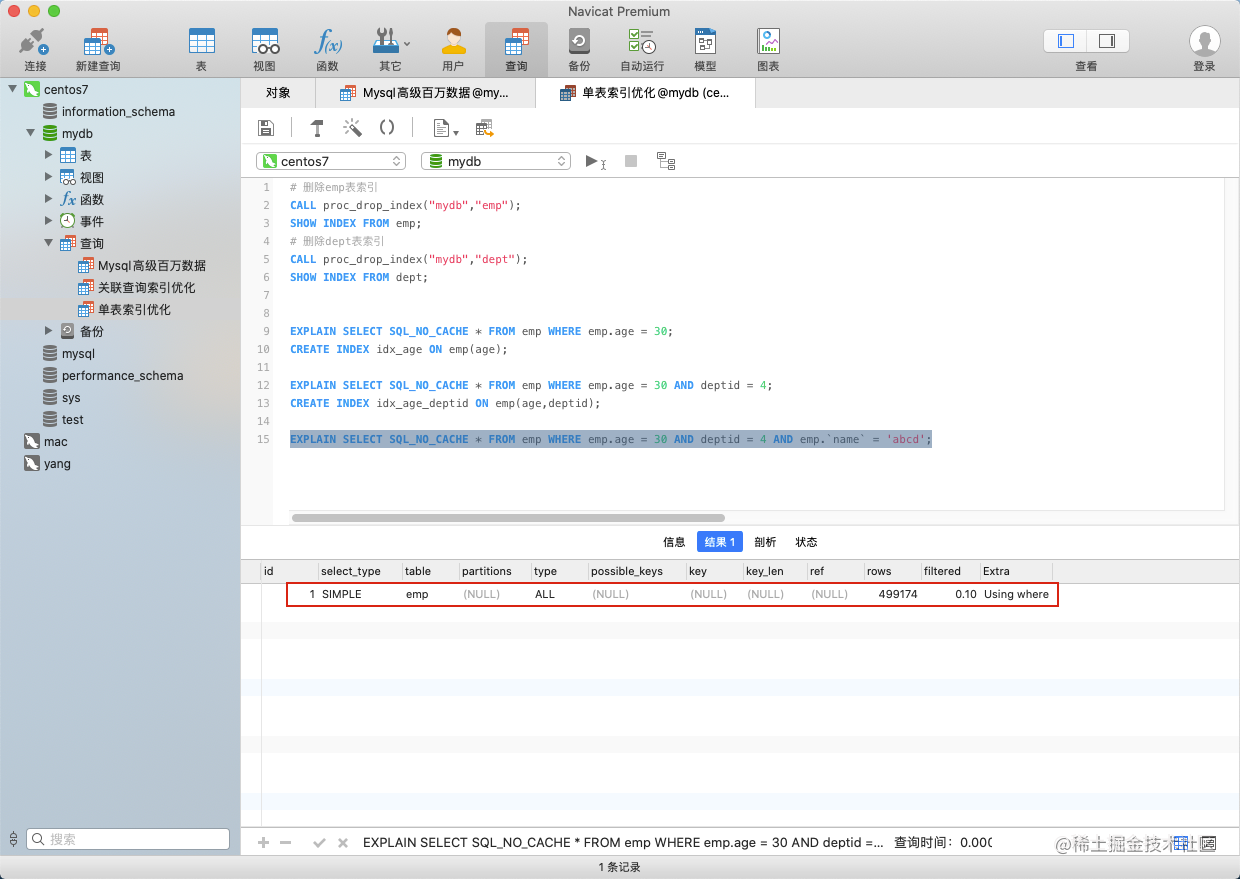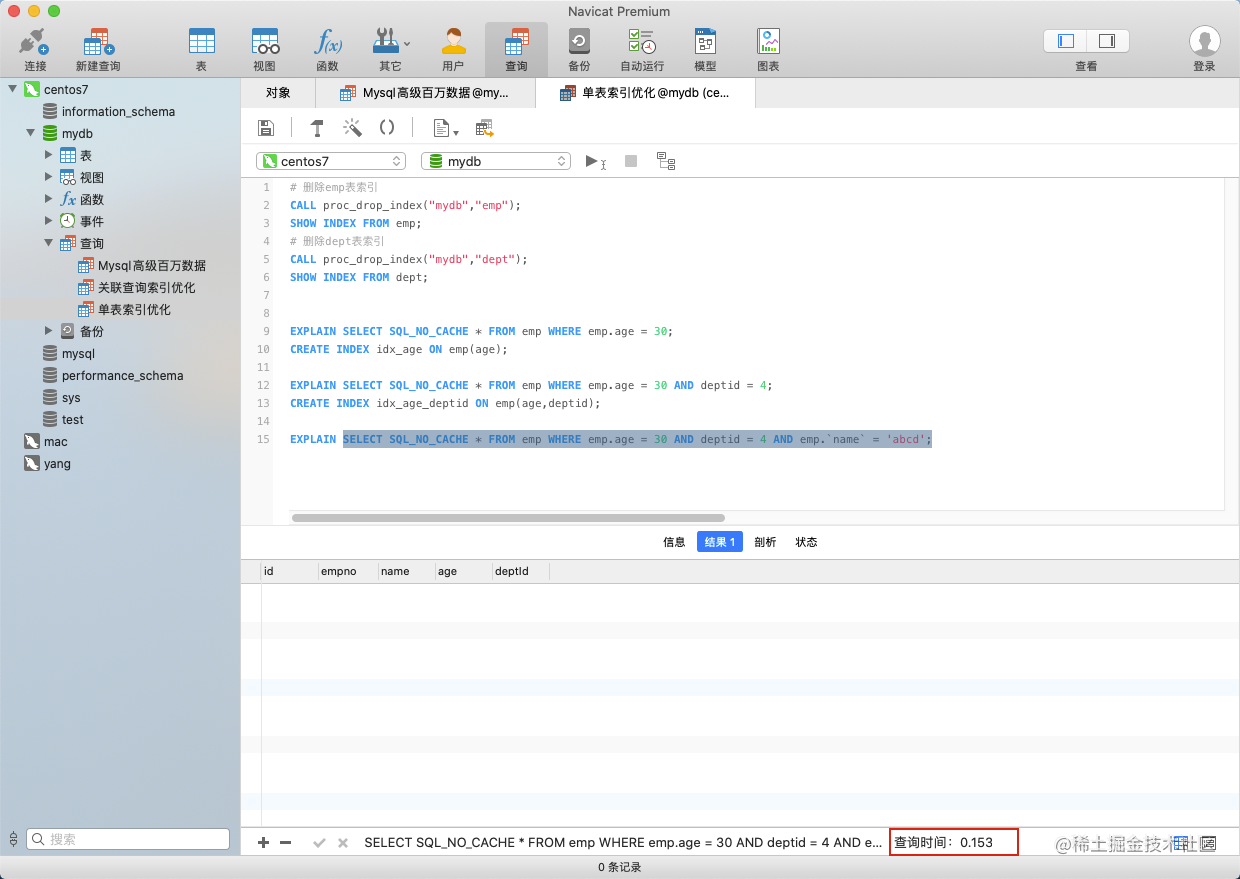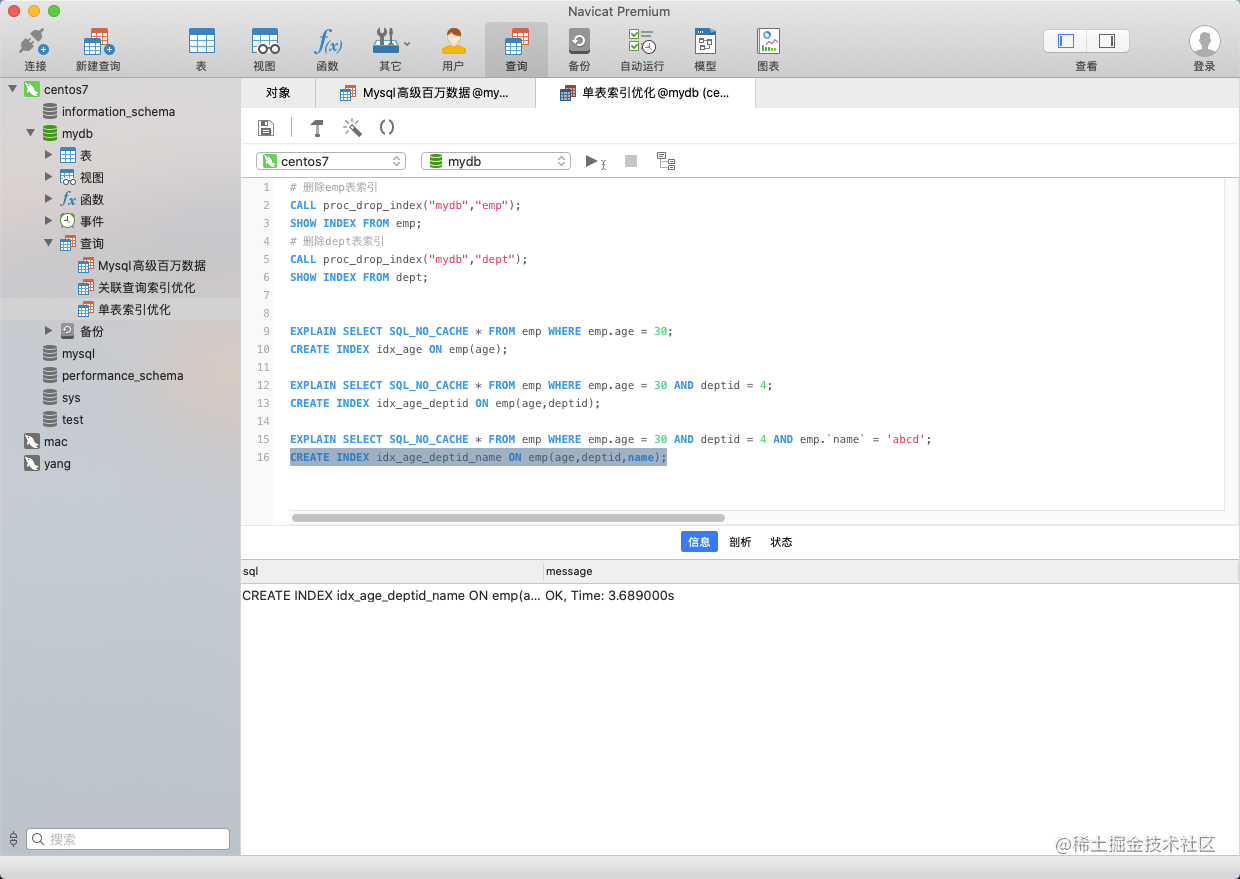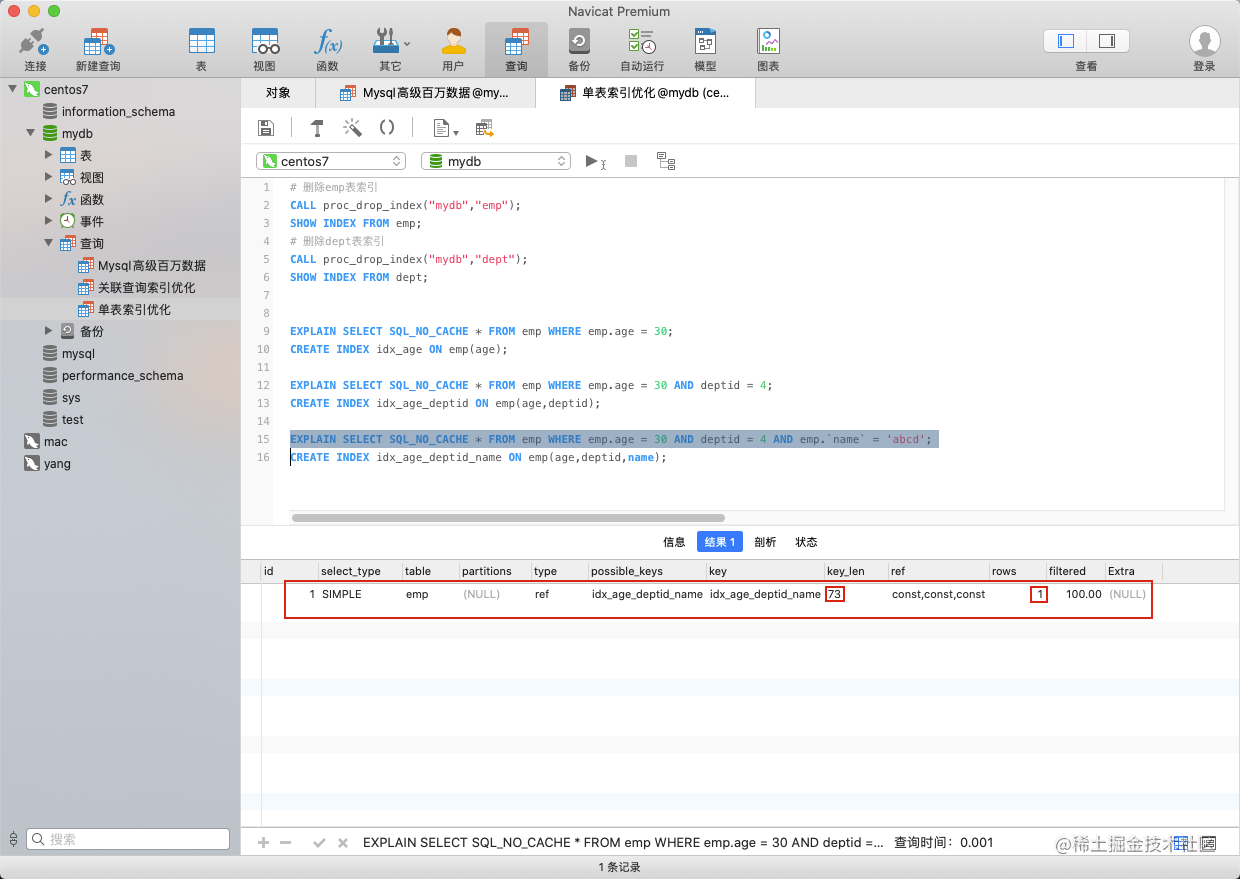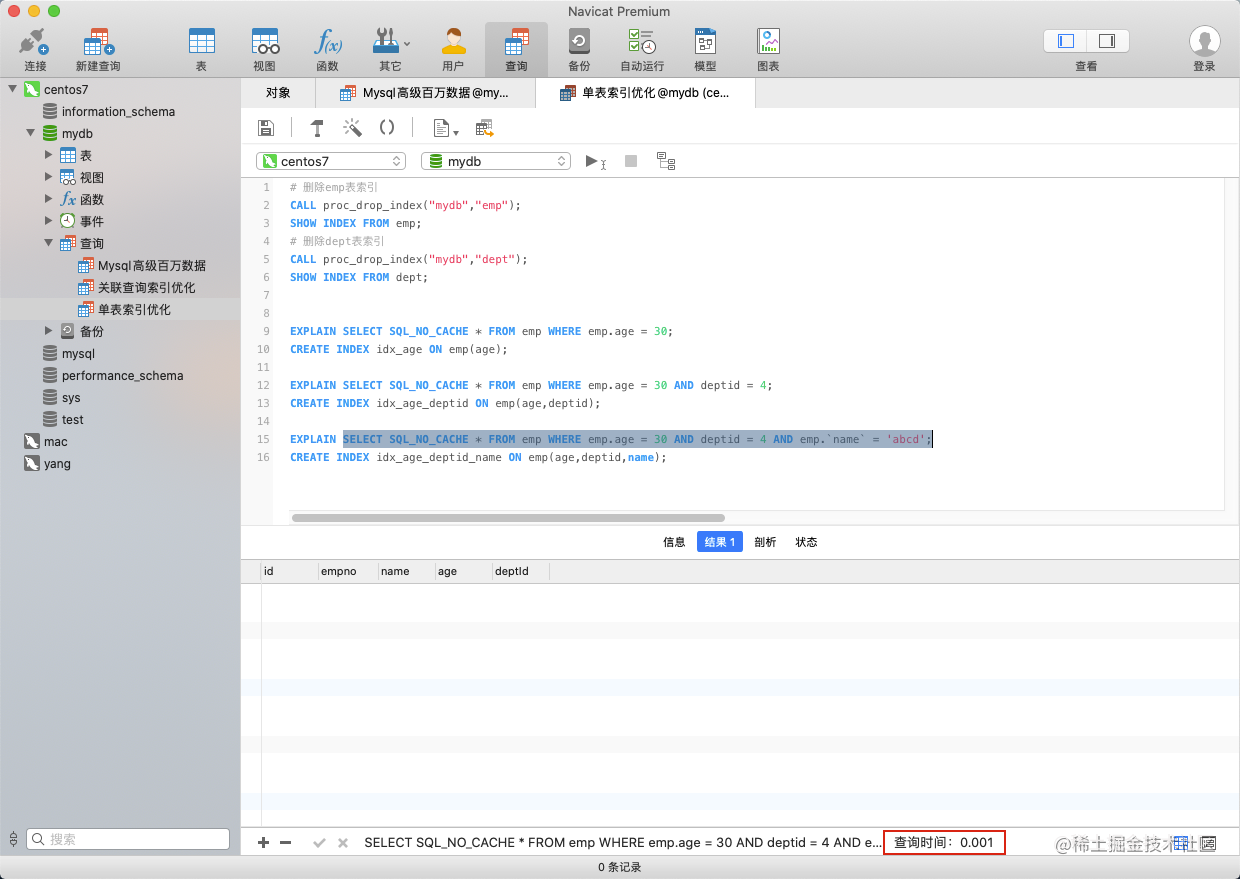``````EXPLAIN SELECT SQL_NO_CACHE * FROM emp WHERE emp.age = 30;
CREATE INDEX idx_age ON emp(age);

EXPLAIN SELECT SQL_NO_CACHE * FROM emp WHERE emp.age = 30 AND deptid = 4;
CREATE INDEX idx_age_deptid ON emp(age,deptid);

EXPLAIN SELECT SQL_NO_CACHE * FROM emp WHERE emp.age = 30 AND deptid = 4 AND emp.`name` = 'abcd';
CREATE INDEX idx_age_deptid_name ON emp(age,deptid,name);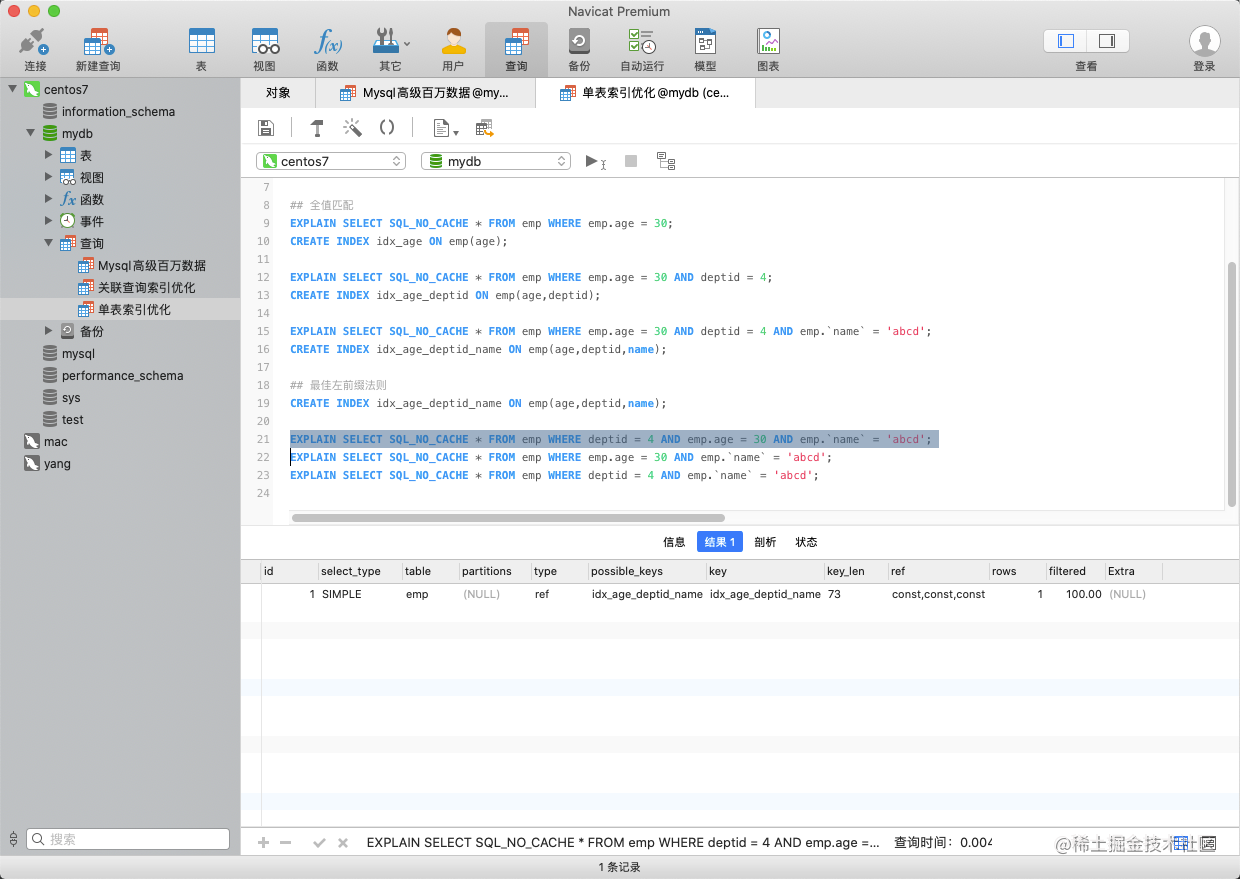`age`条件放到最前后面接着`name`条件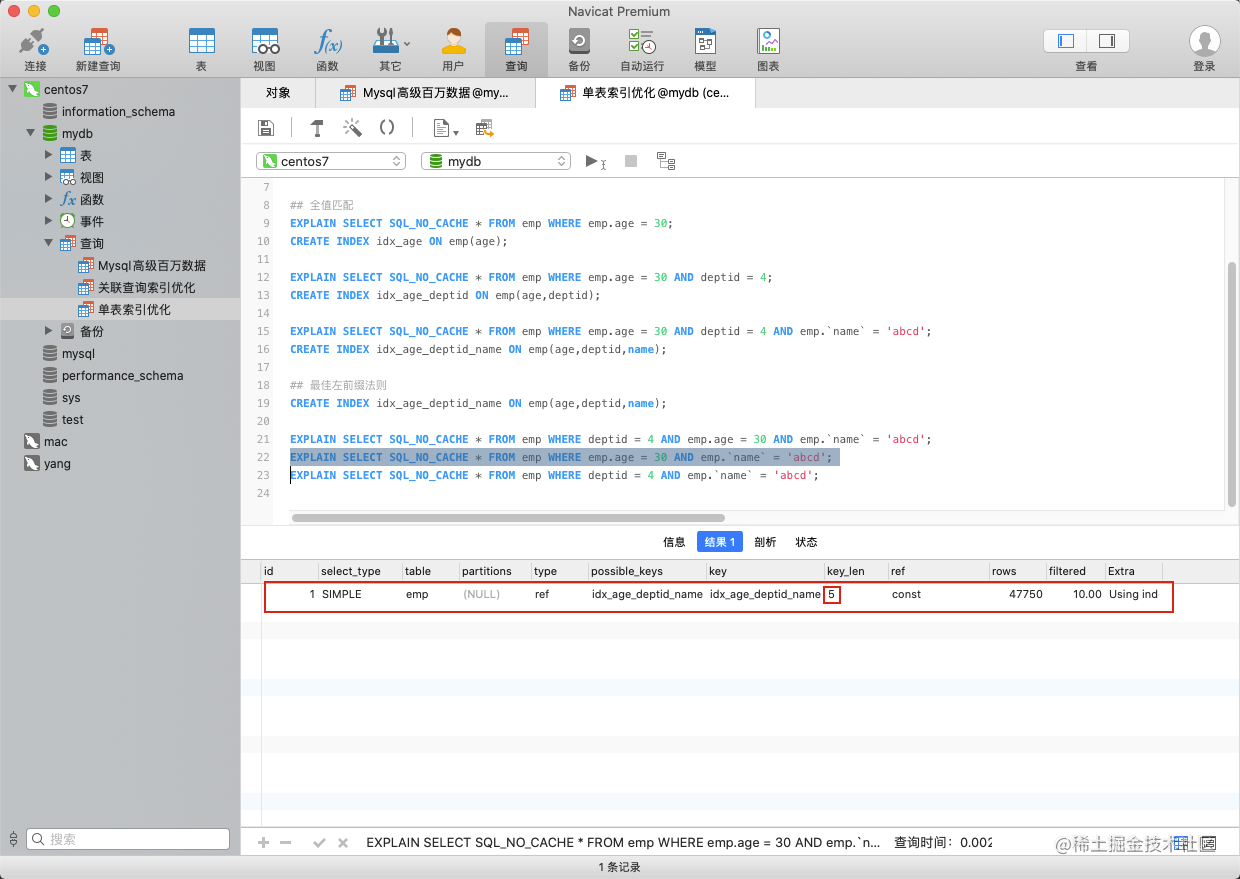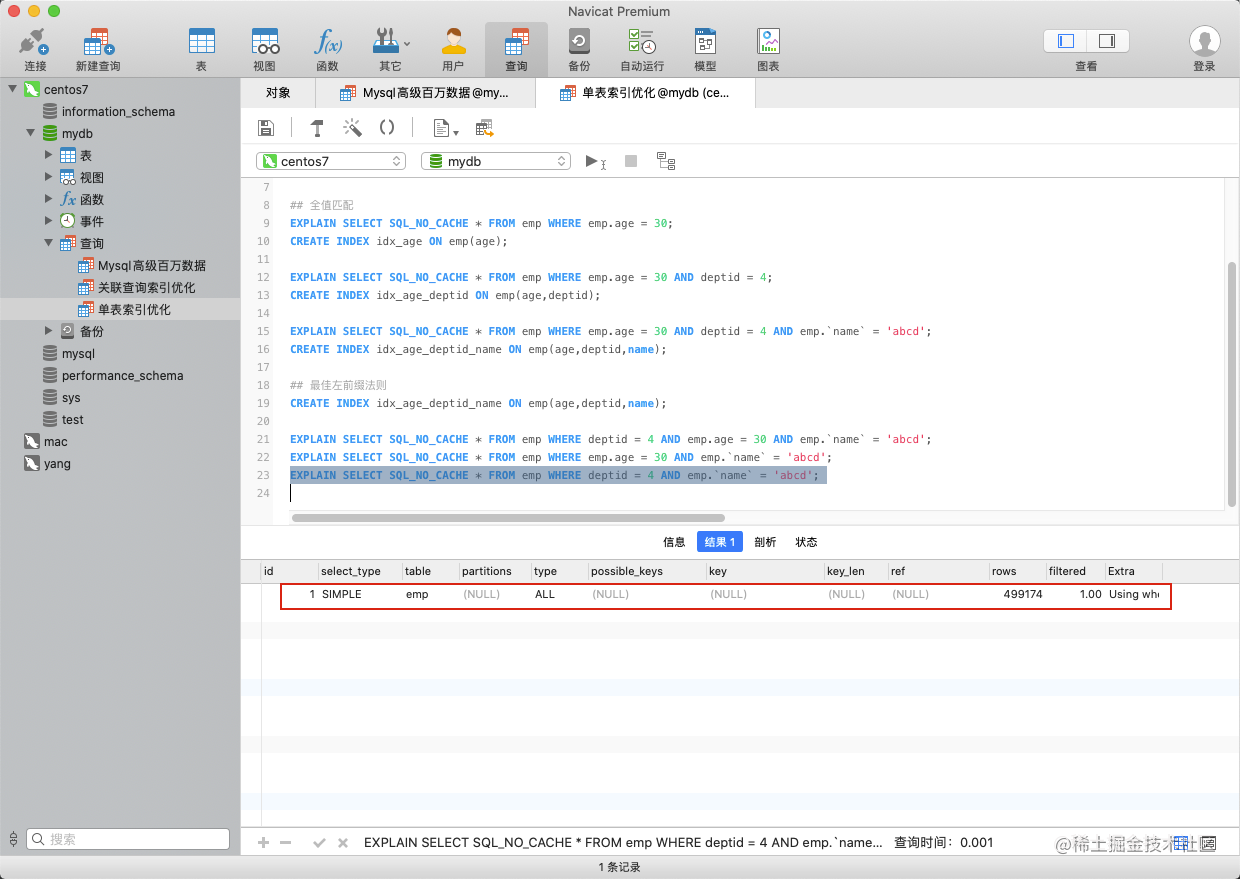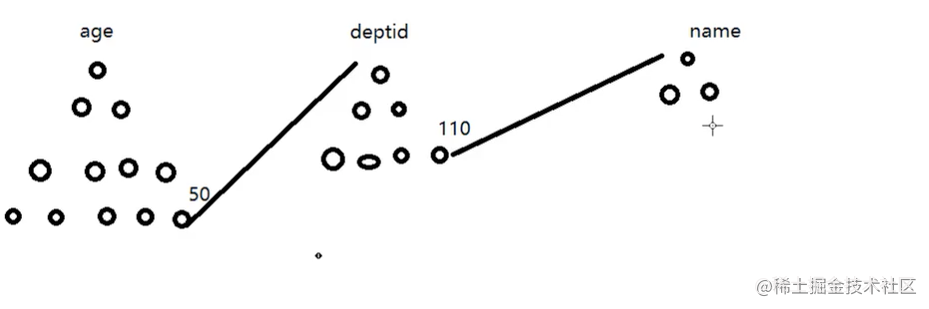``````## 最佳左前缀法则
CREATE INDEX idx_age_deptid_name ON emp(age,deptid,name);

EXPLAIN SELECT SQL_NO_CACHE * FROM emp WHERE deptid = 4 AND emp.age = 30 AND emp.`name` = 'abcd';
EXPLAIN SELECT SQL_NO_CACHE * FROM emp WHERE emp.age = 30 AND emp.`name` = 'abcd';
EXPLAIN SELECT SQL_NO_CACHE * FROM emp WHERE deptid = 4 AND emp.`name` = 'abcd';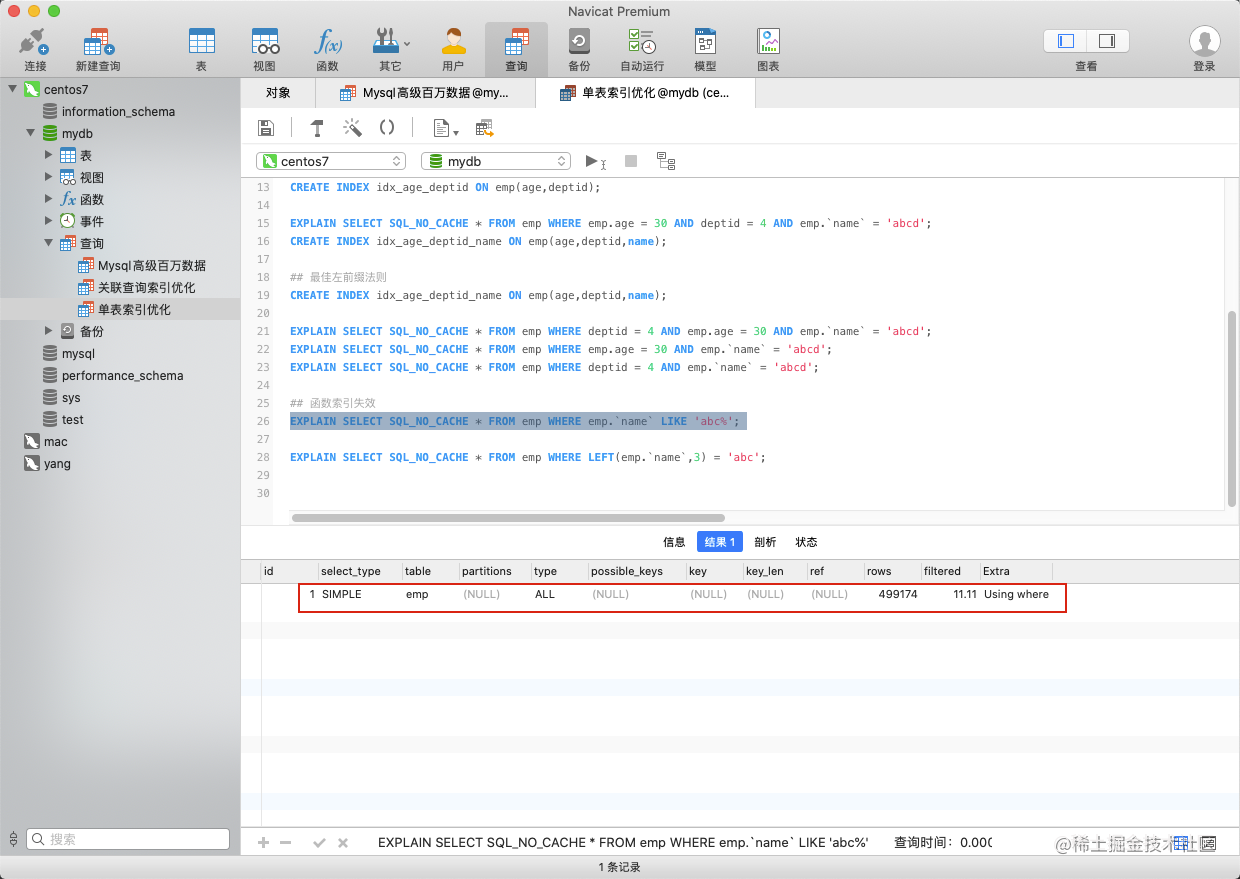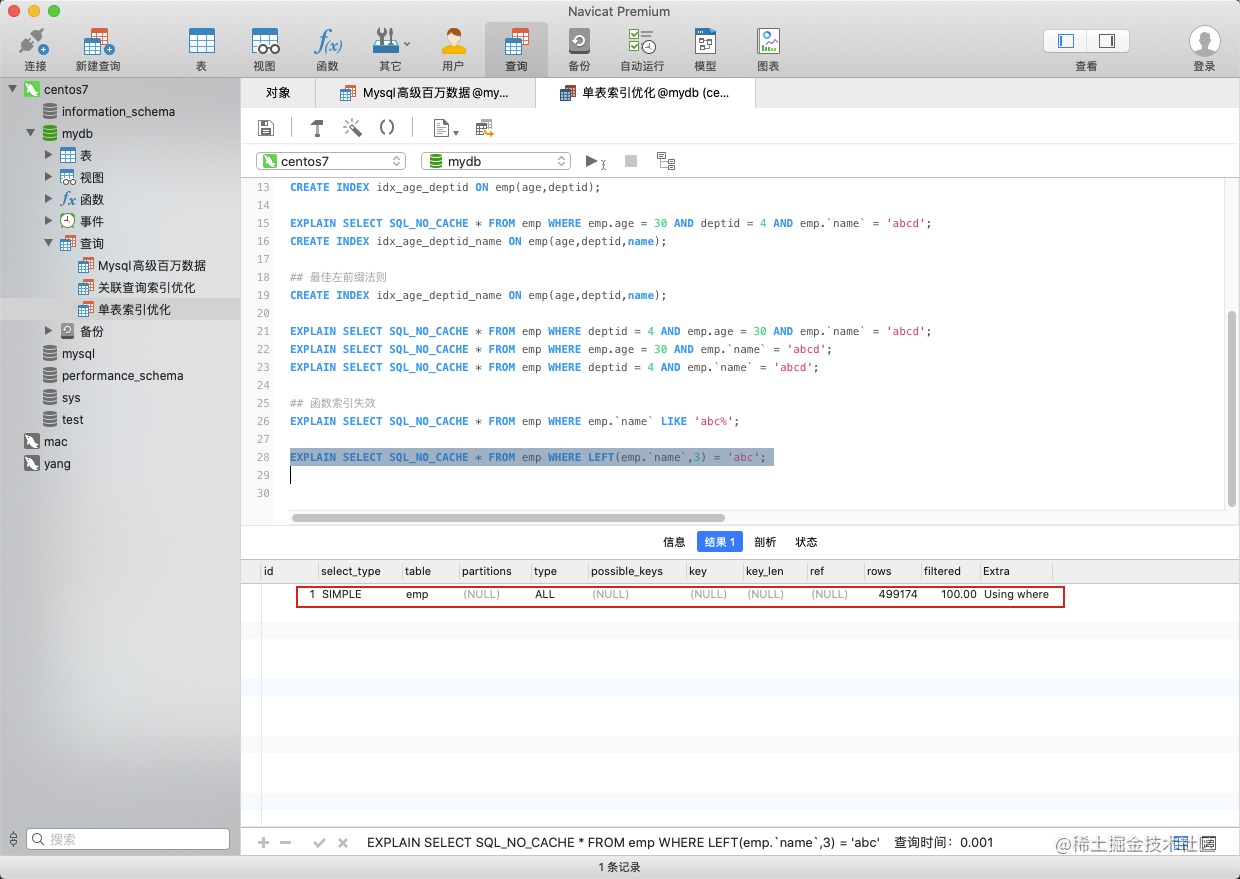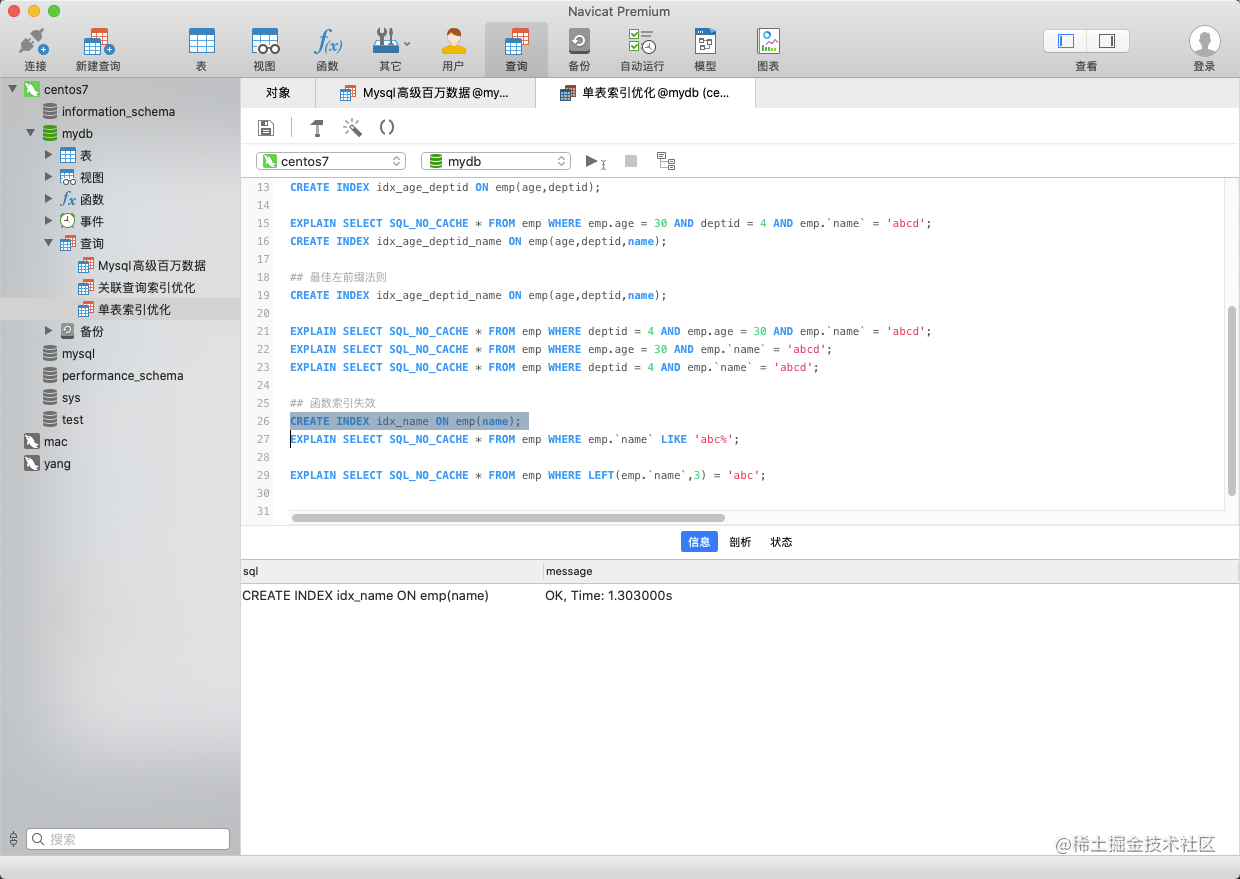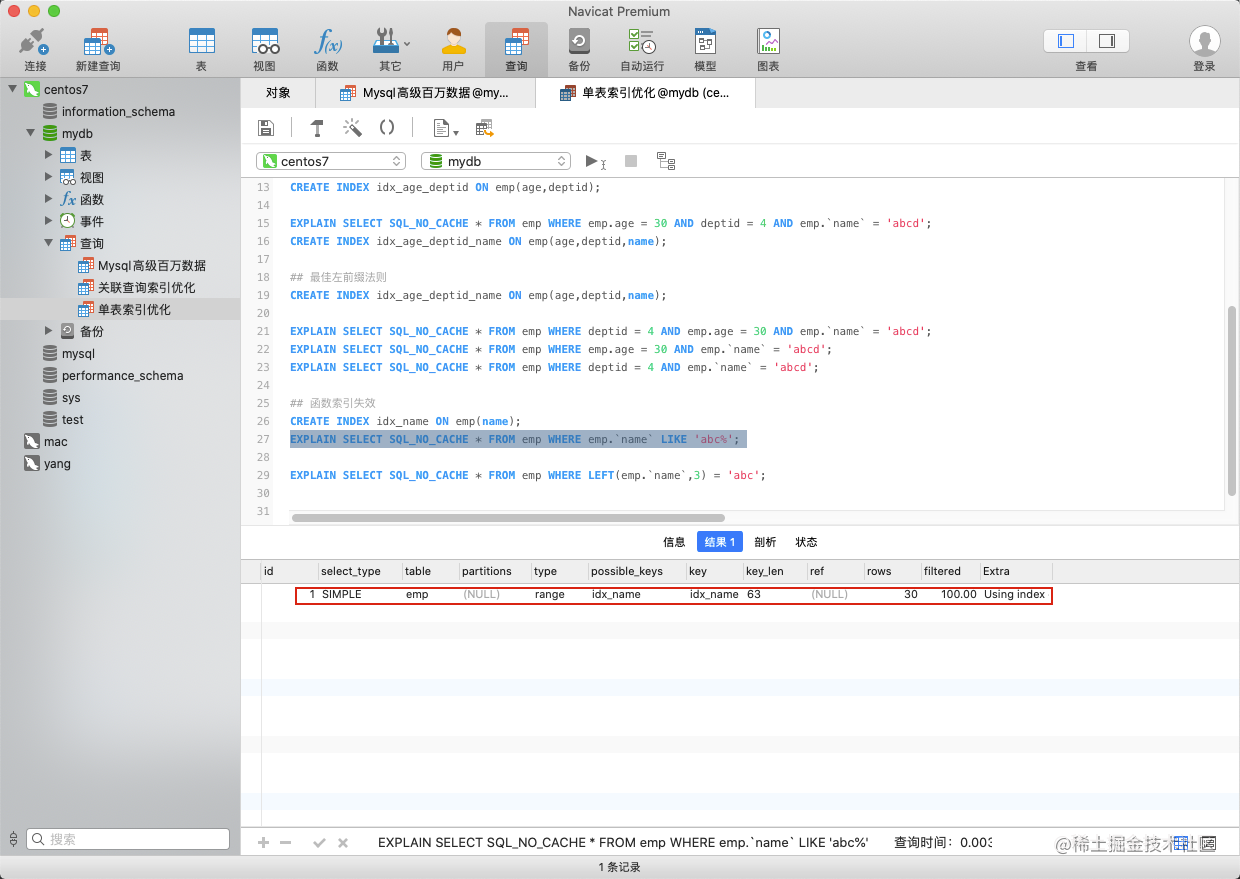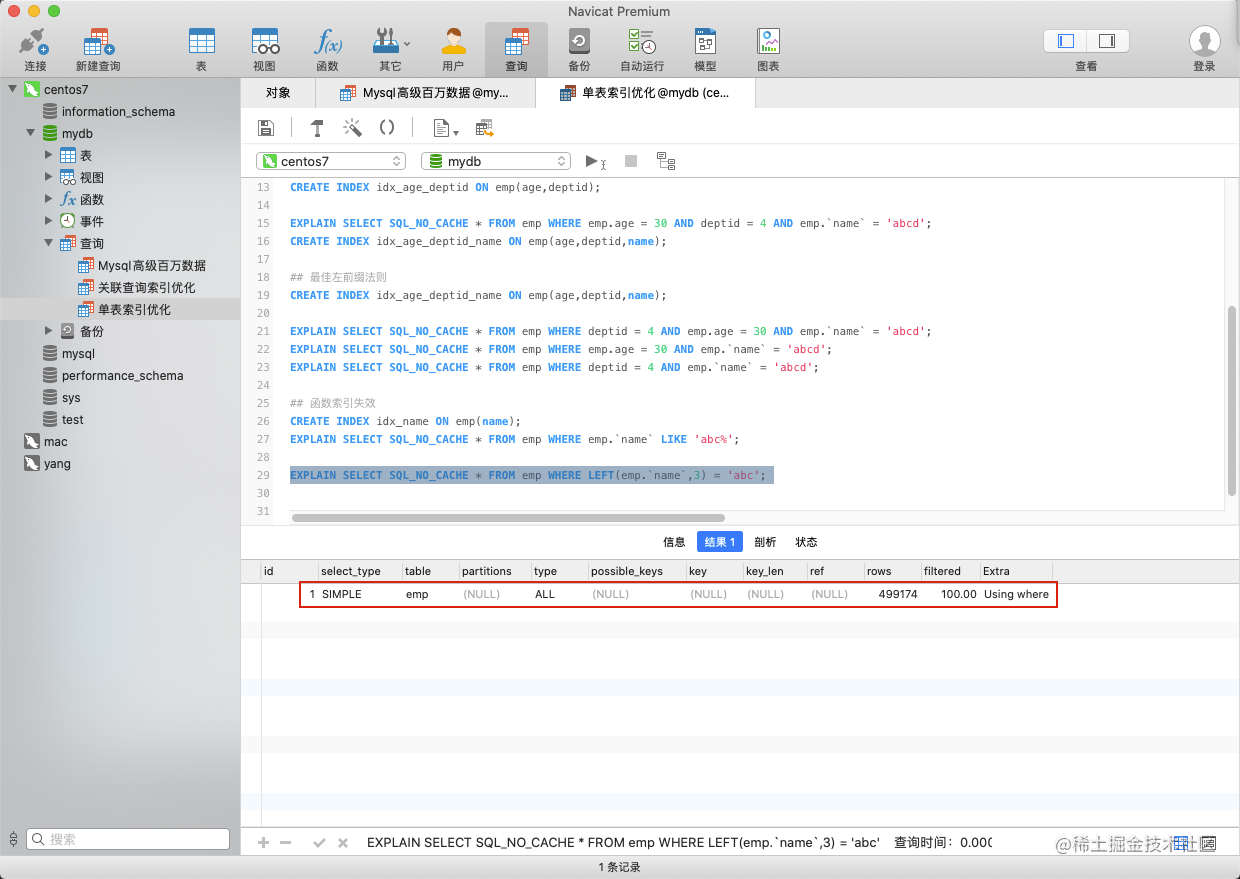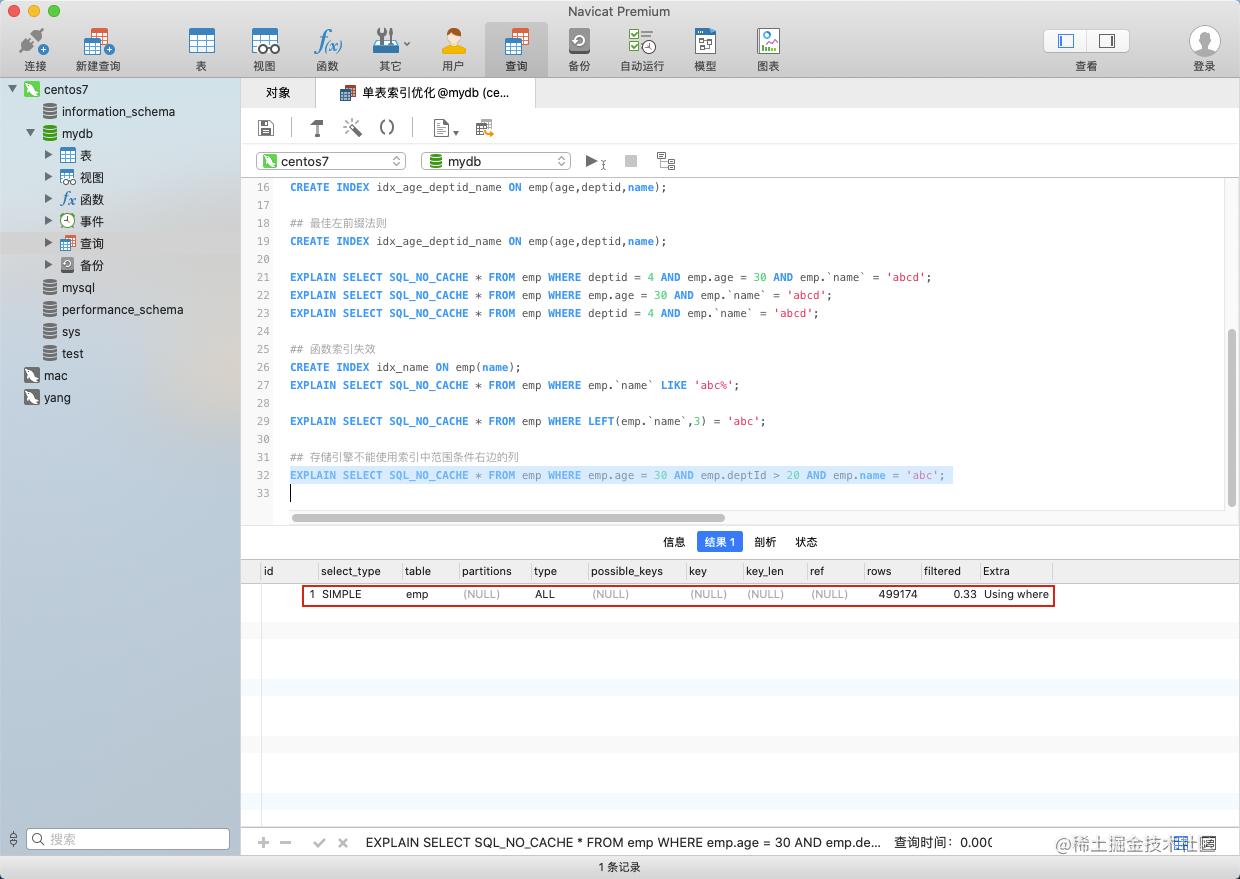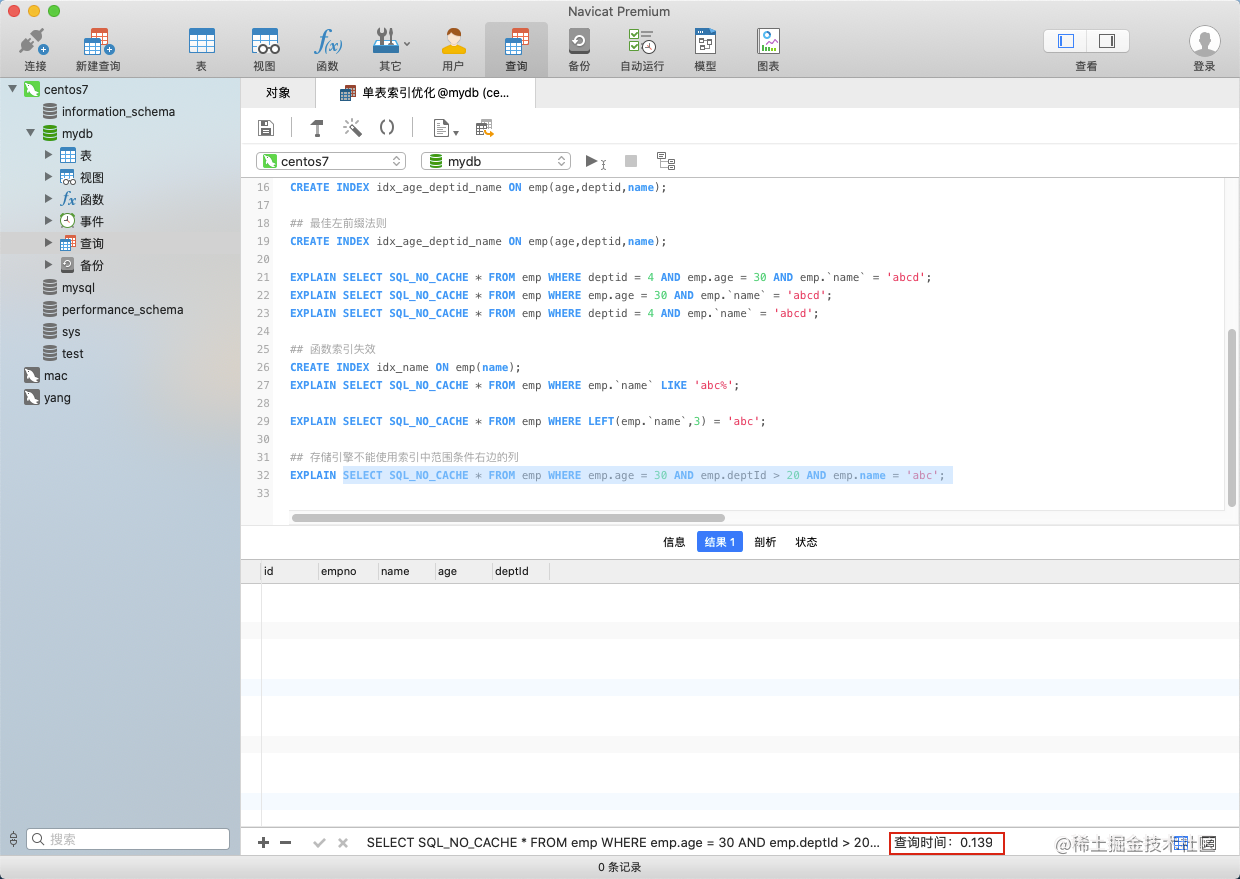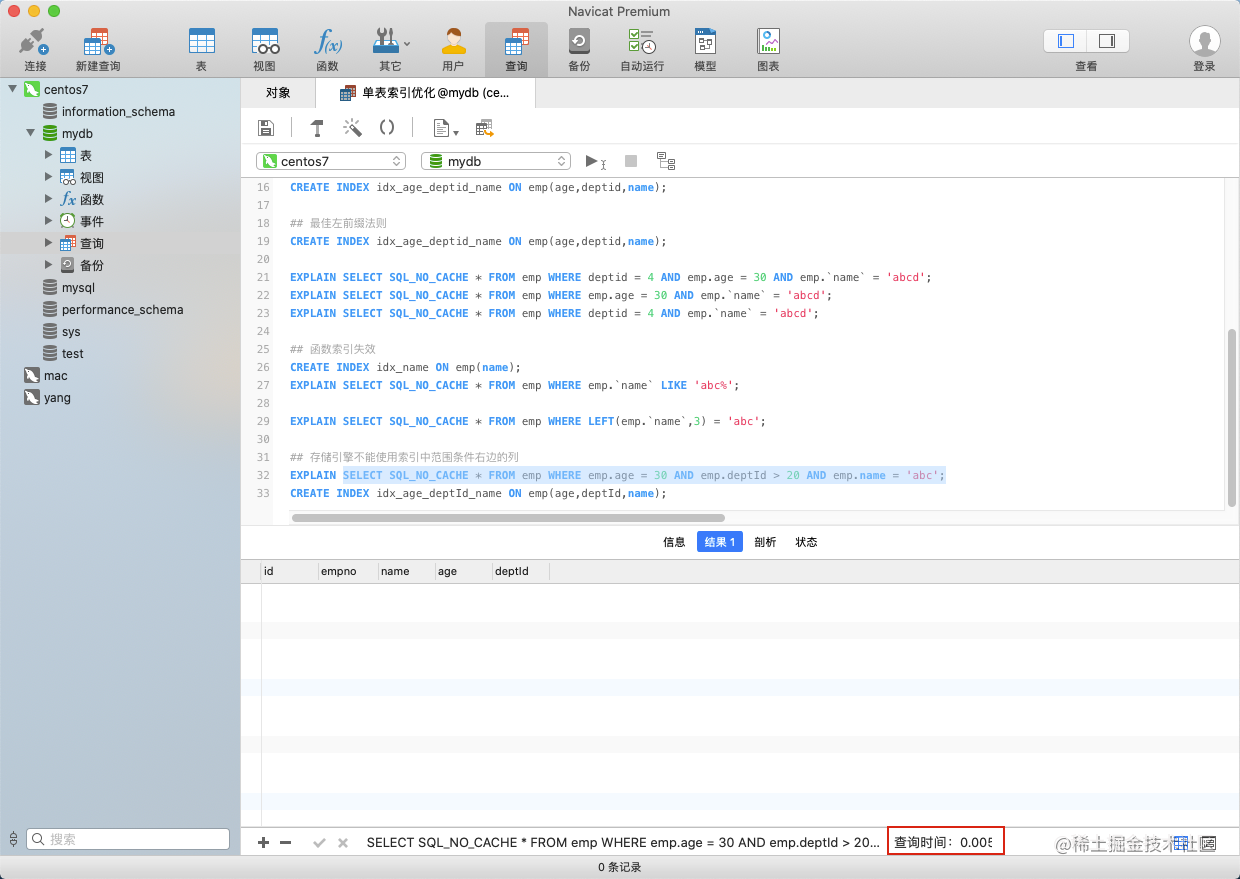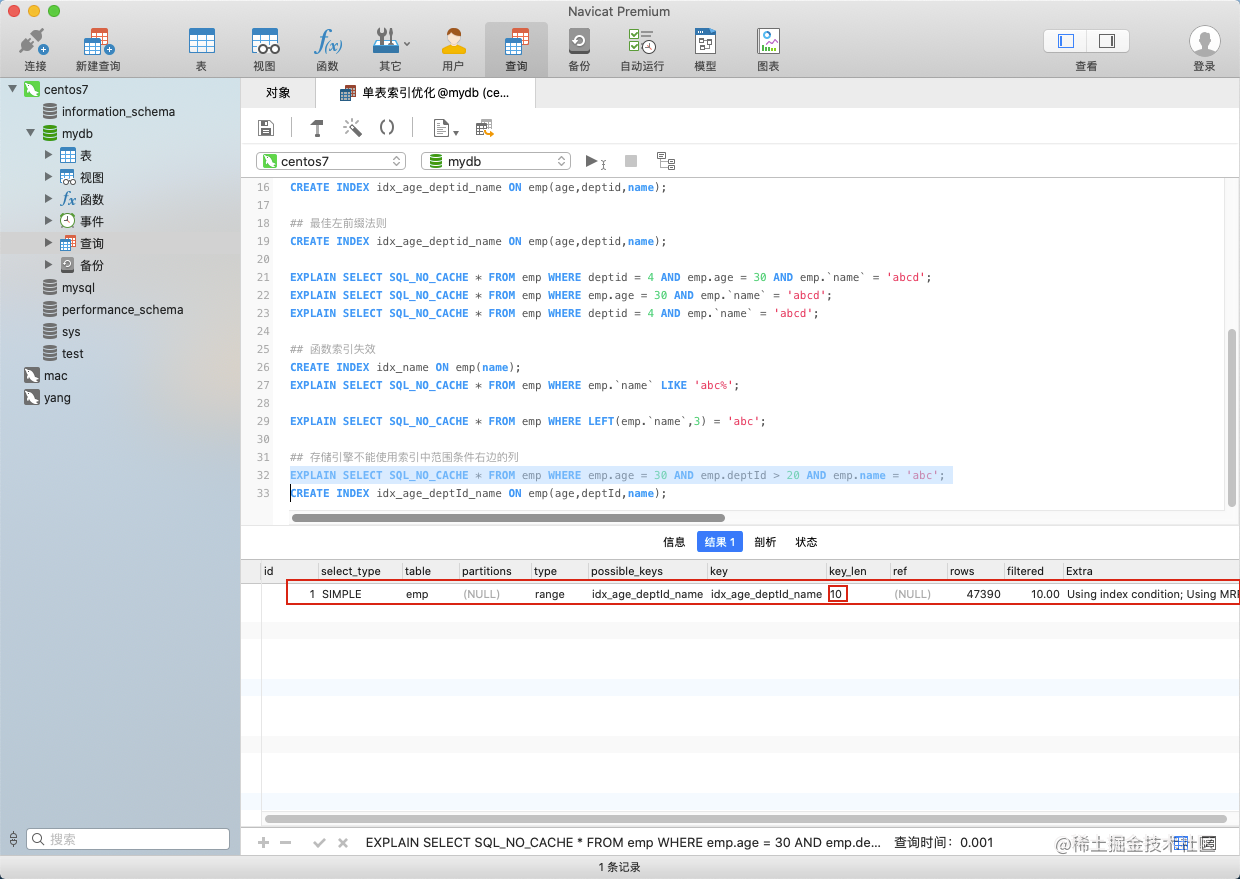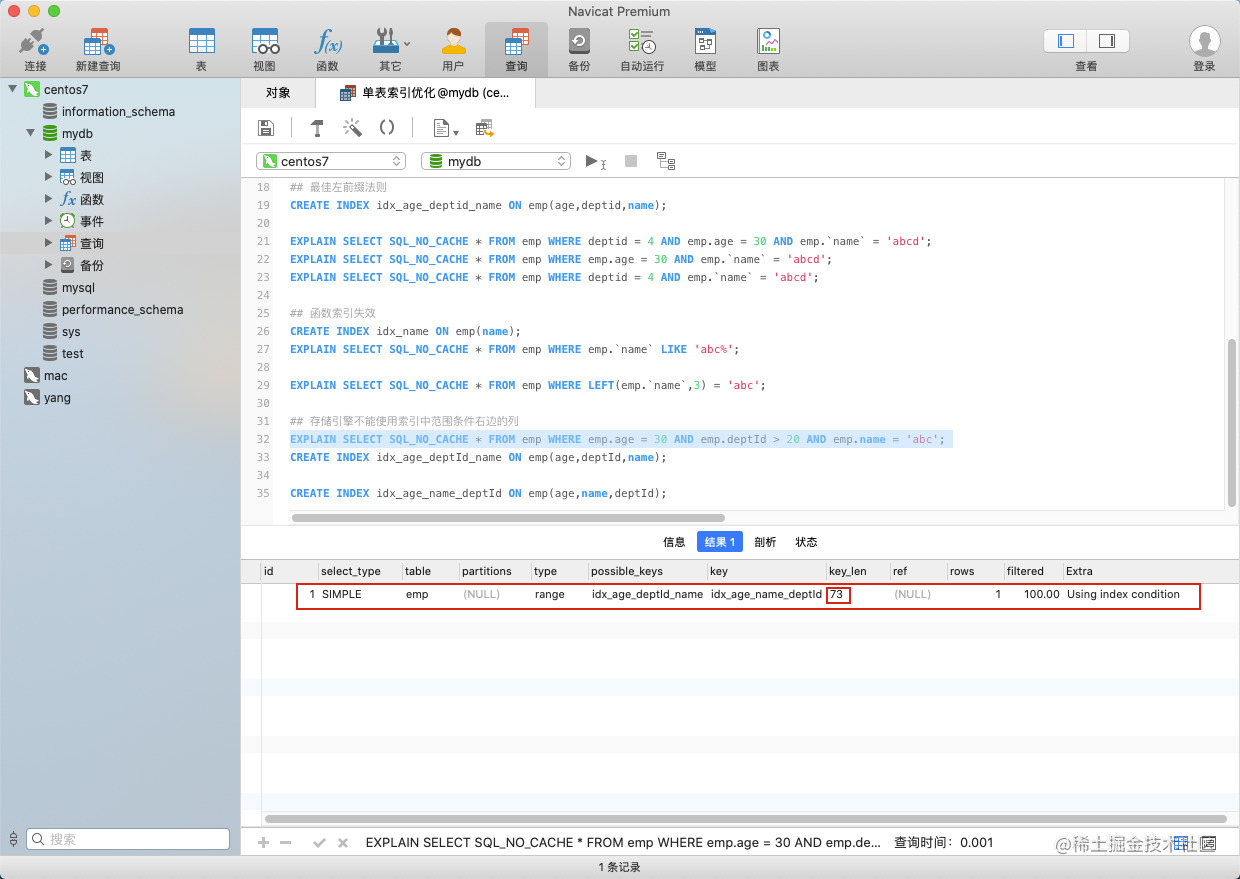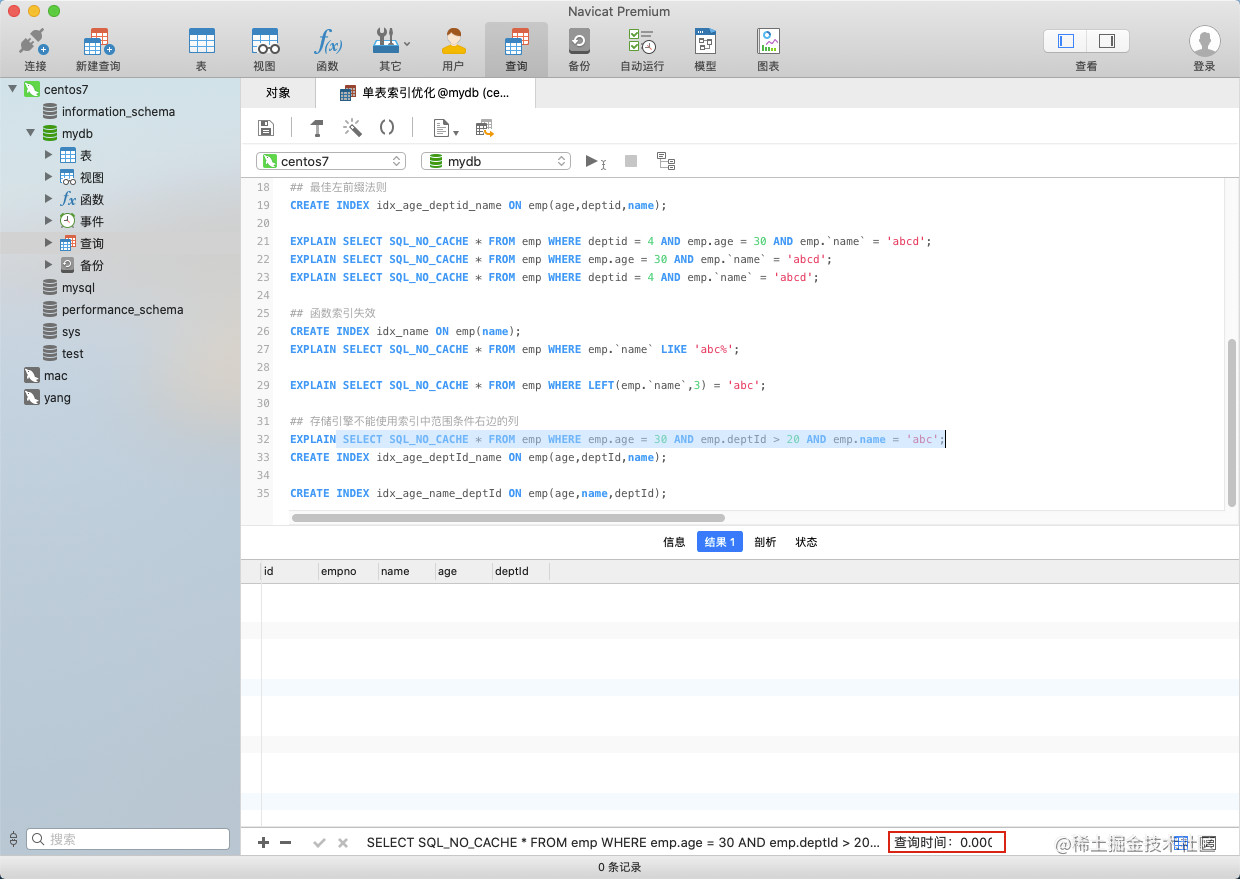``````## 存储引擎不能使用索引中范围条件右边的列
EXPLAIN SELECT SQL_NO_CACHE * FROM emp WHERE emp.age = 30 AND emp.deptId > 20 AND emp.name = 'abc';
CREATE INDEX idx_age_deptId_name ON emp(age,deptId,name);

CREATE INDEX idx_age_name_deptId ON emp(age,name,deptId);

mysql在使用不等于(!= 或者<>)的时候无法使用索引会导致全表扫描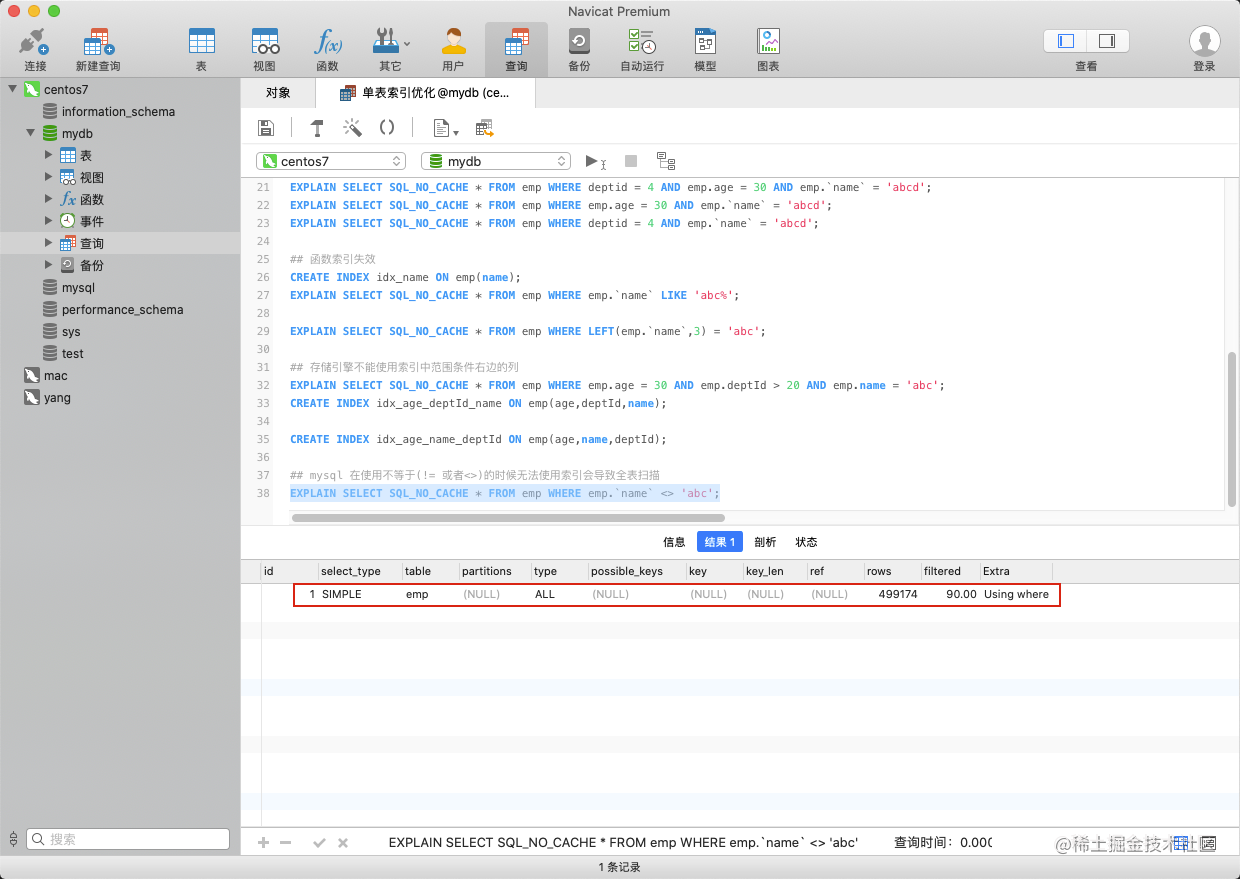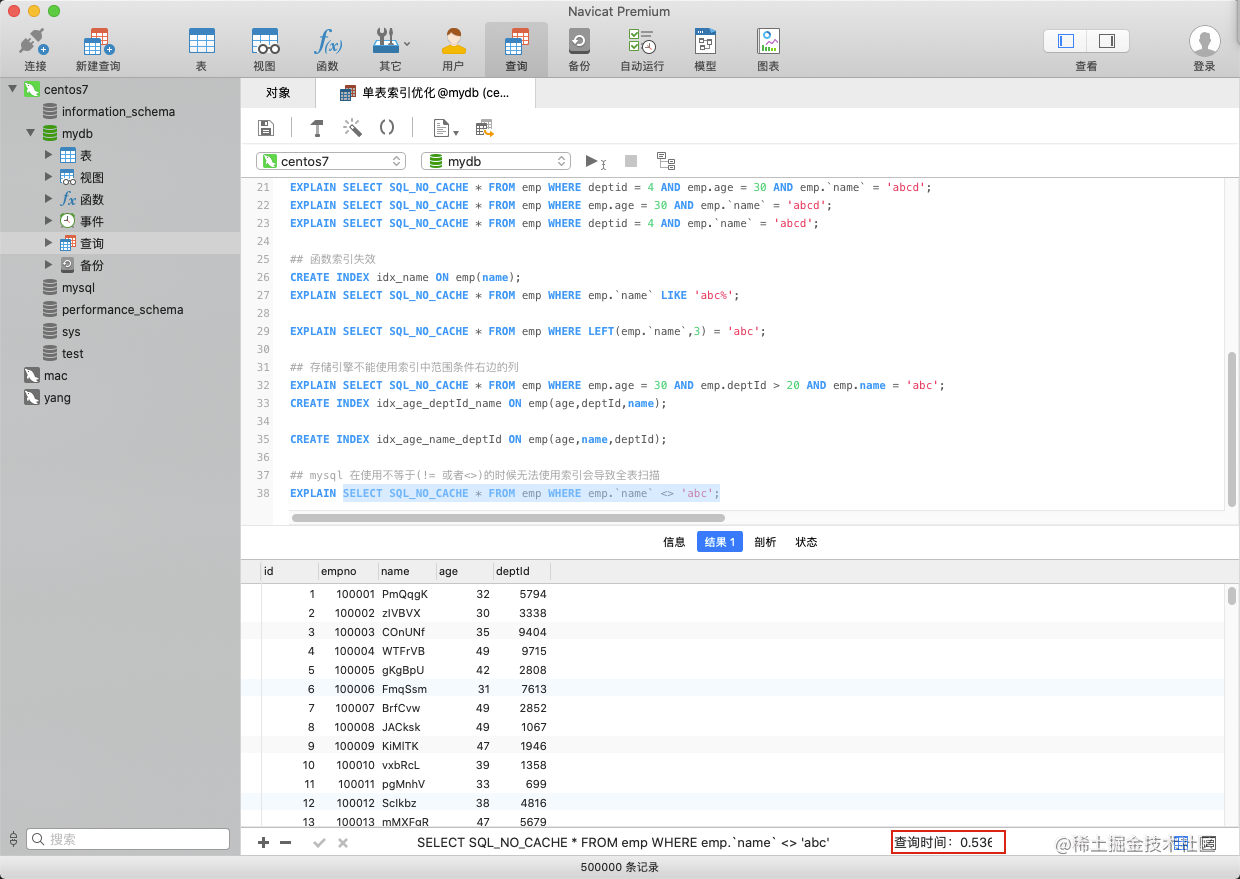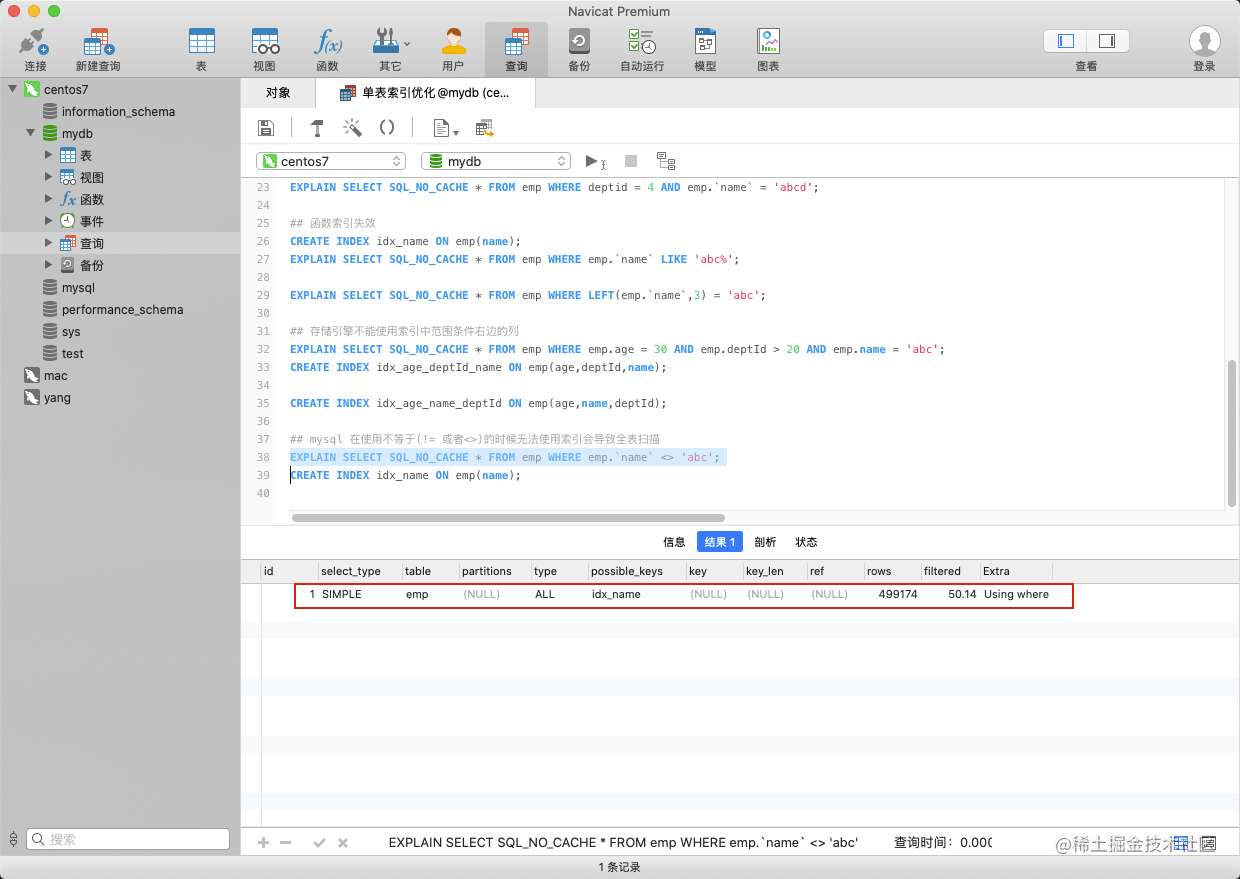mysql在使用不等于(!= 或者<>)的时候无法使用索引会导致全表扫描

``````## mysql 在使用不等于(!= 或者<>)的时候无法使用索引会导致全表扫描
EXPLAIN SELECT SQL_NO_CACHE * FROM emp WHERE emp.`name` <> 'abc';
CREATE INDEX idx_name ON emp(name);

`is null`是可以使用索引的,`is not null `无法使用索引。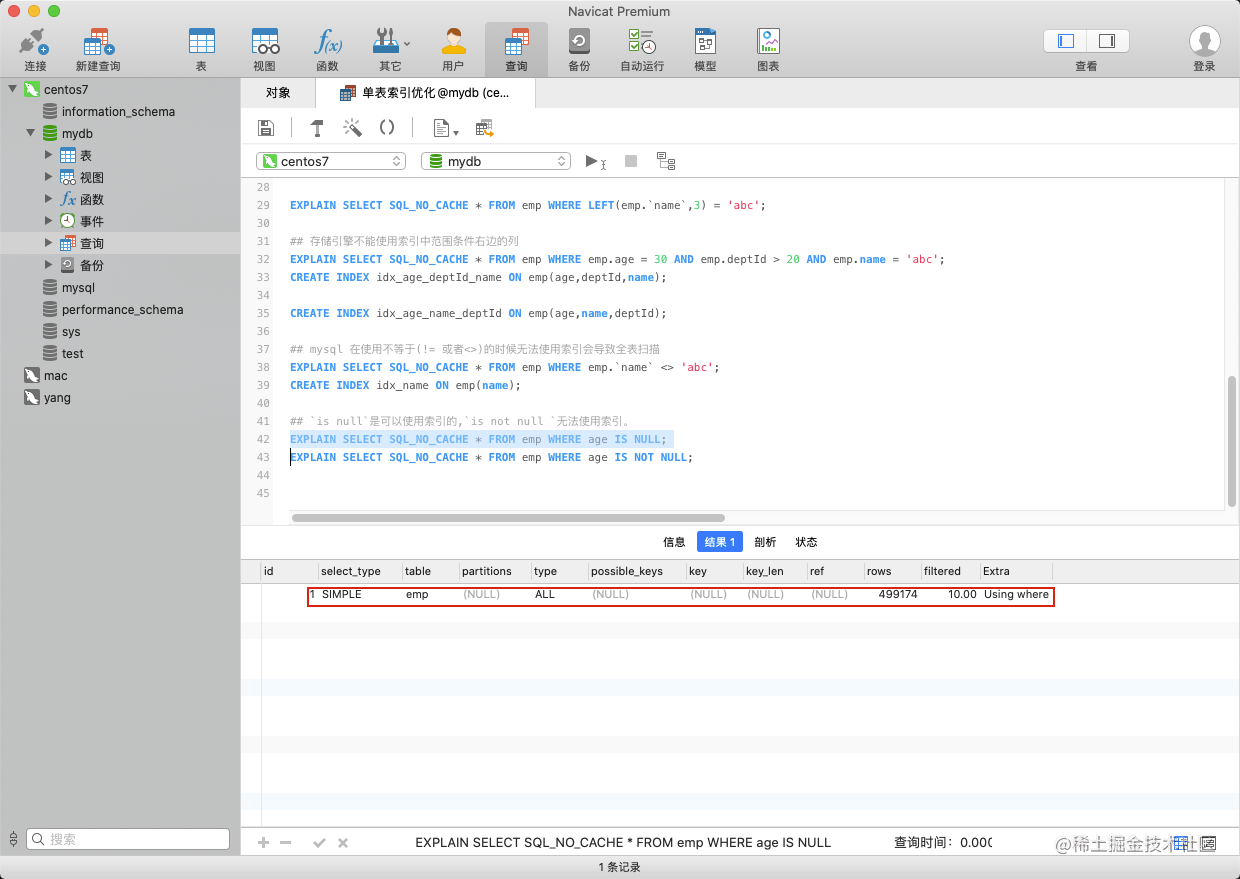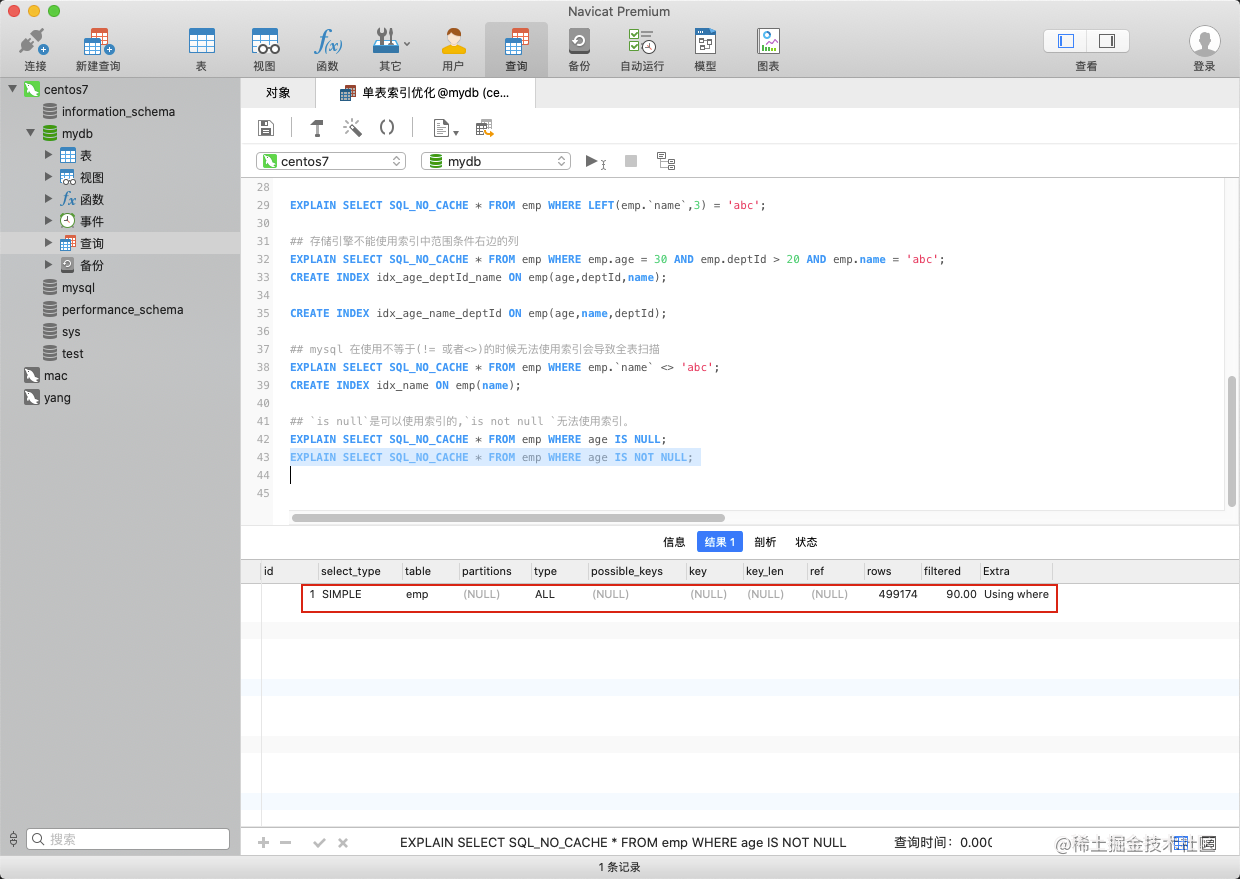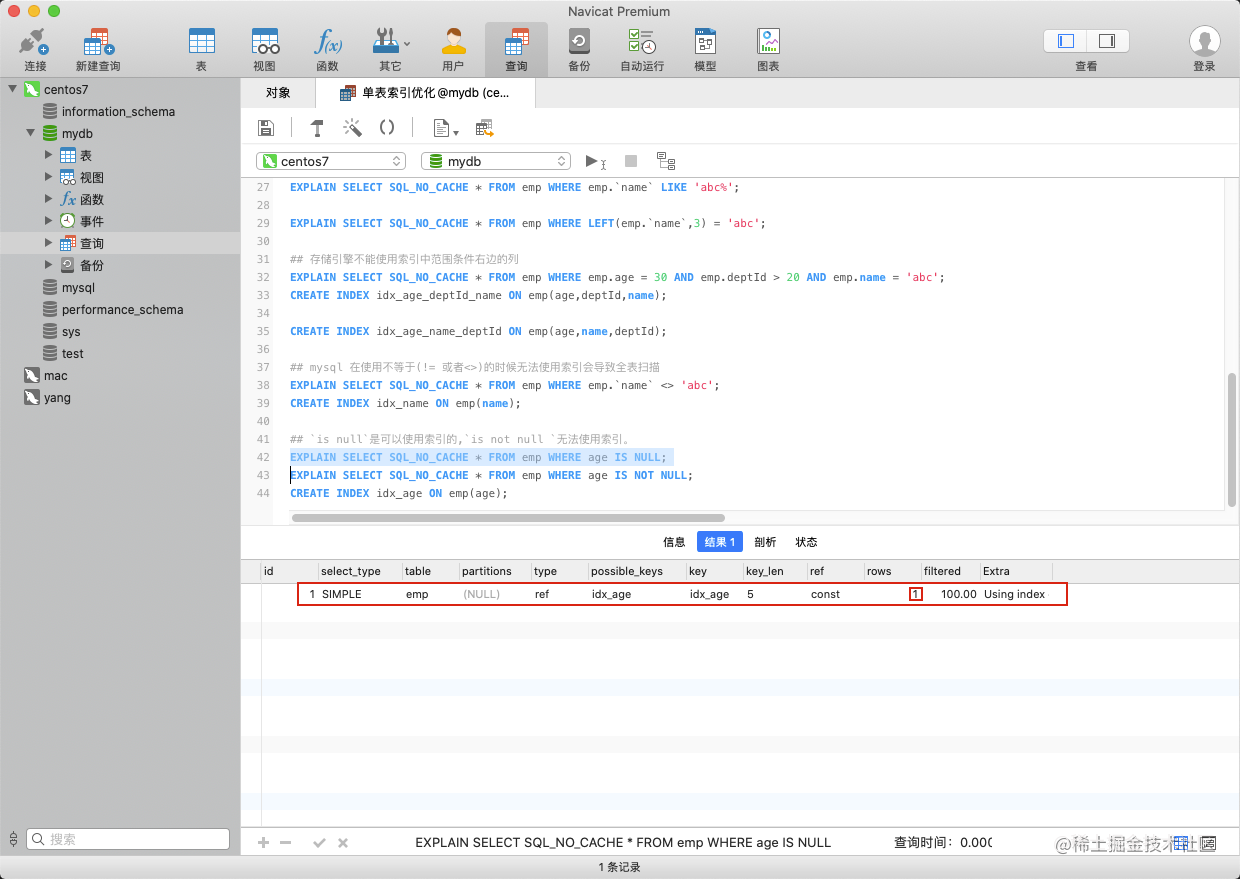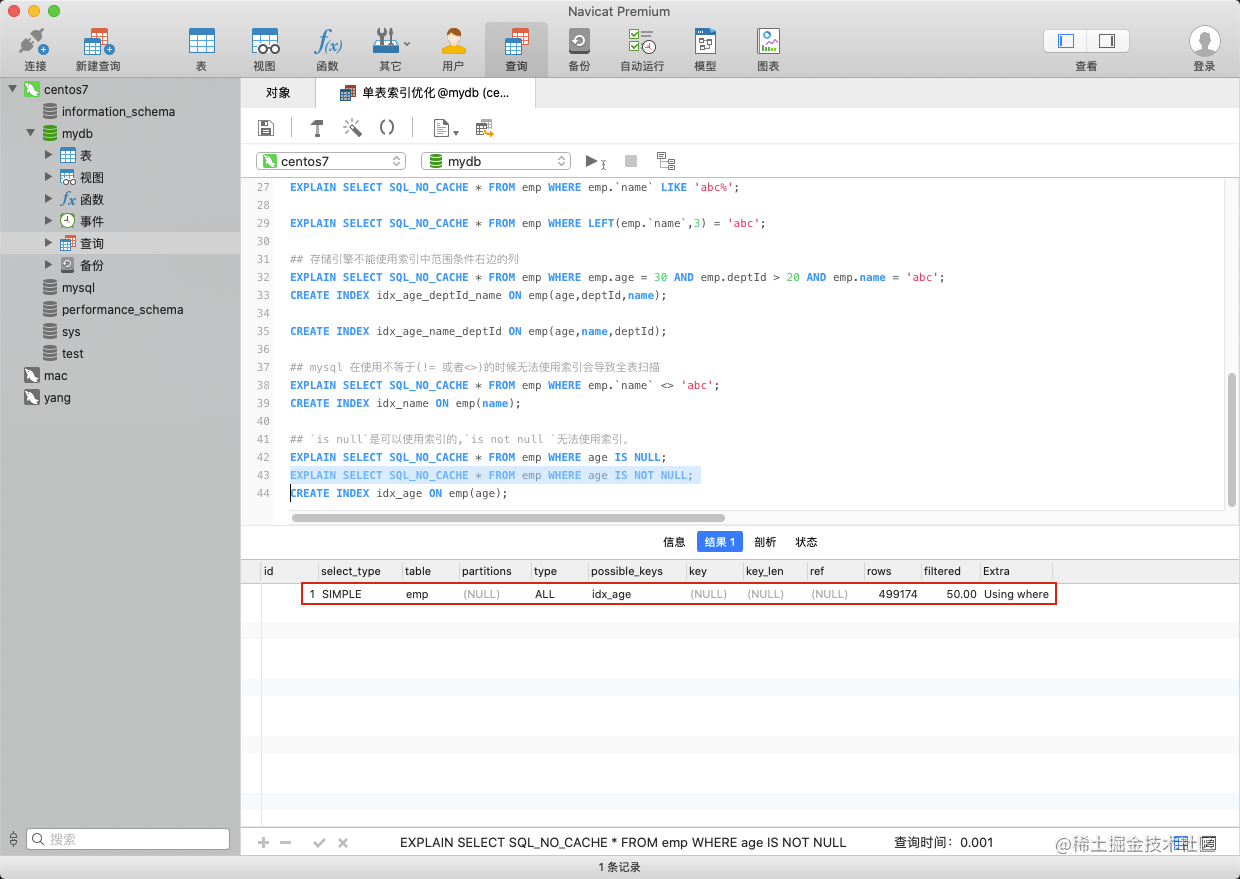`is null`是可以使用索引的,`is not null `无法使用索引。

``````## `is null`是可以使用索引的,`is not null `无法使用索引。
EXPLAIN SELECT SQL_NO_CACHE * FROM emp WHERE age IS NULL;
EXPLAIN SELECT SQL_NO_CACHE * FROM emp WHERE age IS NOT NULL;
CREATE INDEX idx_age ON emp(age);

like以通配符开头('%abc...')mysql索引失效会变成全表扫描的操作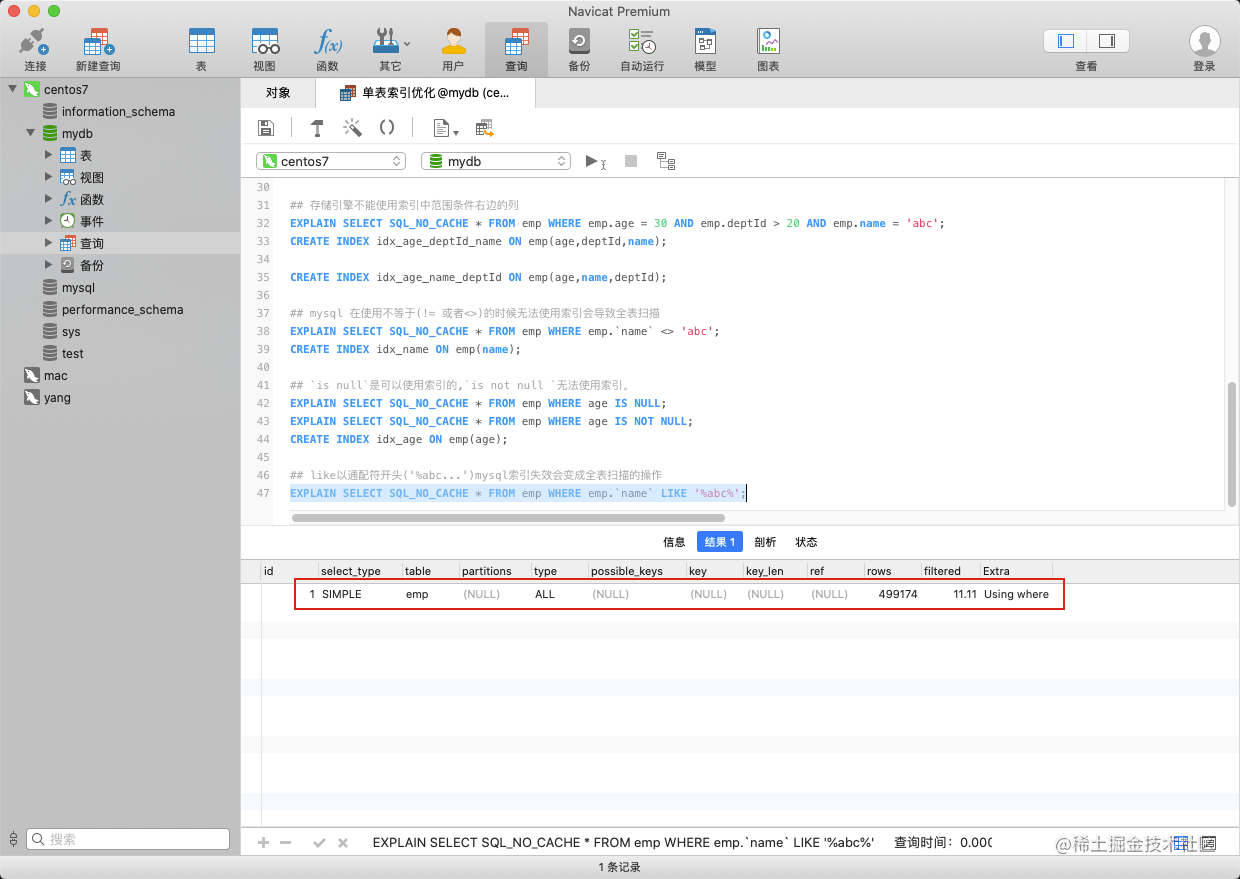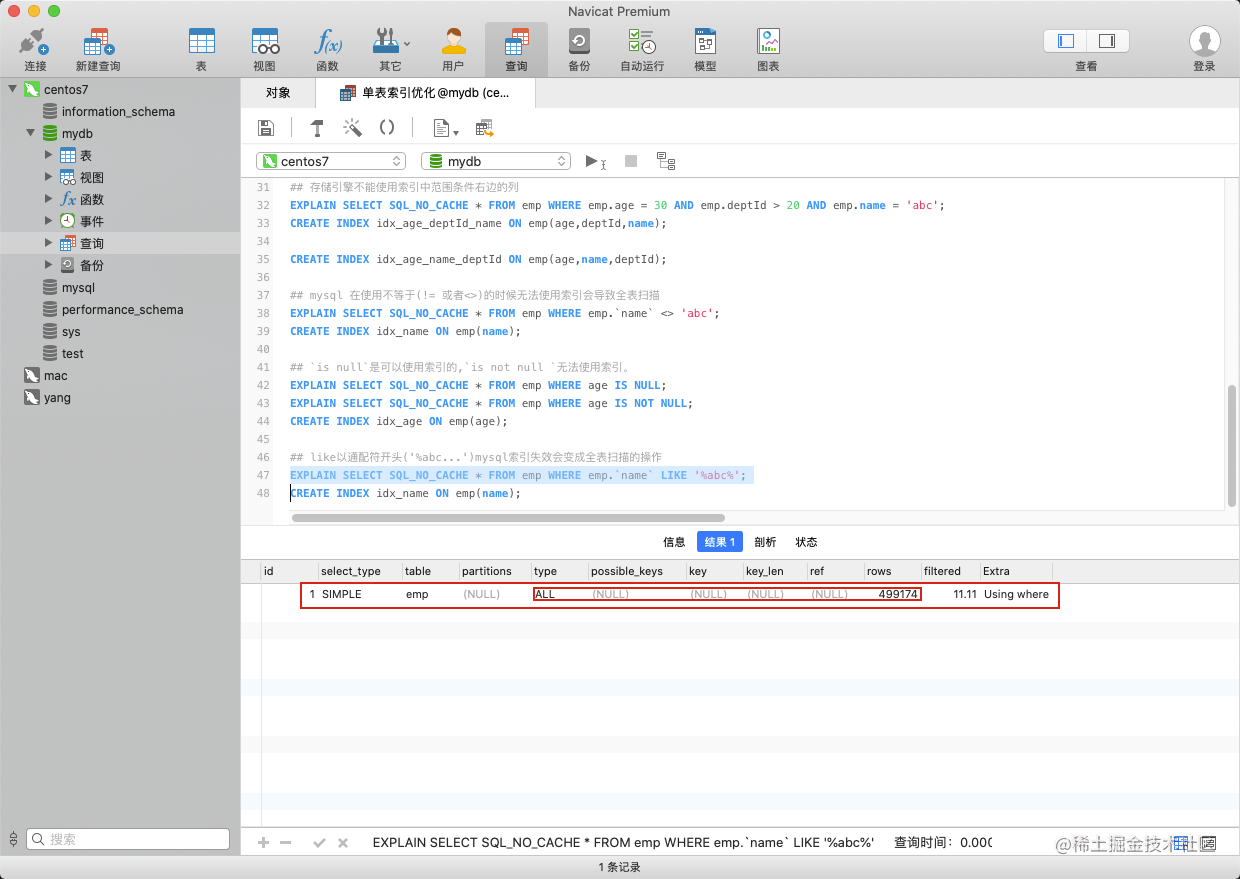like以通配符开头('%abc...')mysql索引失效会变成全表扫描的操作

``````## like以通配符开头('%abc...')mysql索引失效会变成全表扫描的操作
EXPLAIN SELECT SQL_NO_CACHE * FROM emp WHERE emp.`name` LIKE '%abc%';
CREATE INDEX idx_name ON emp(name);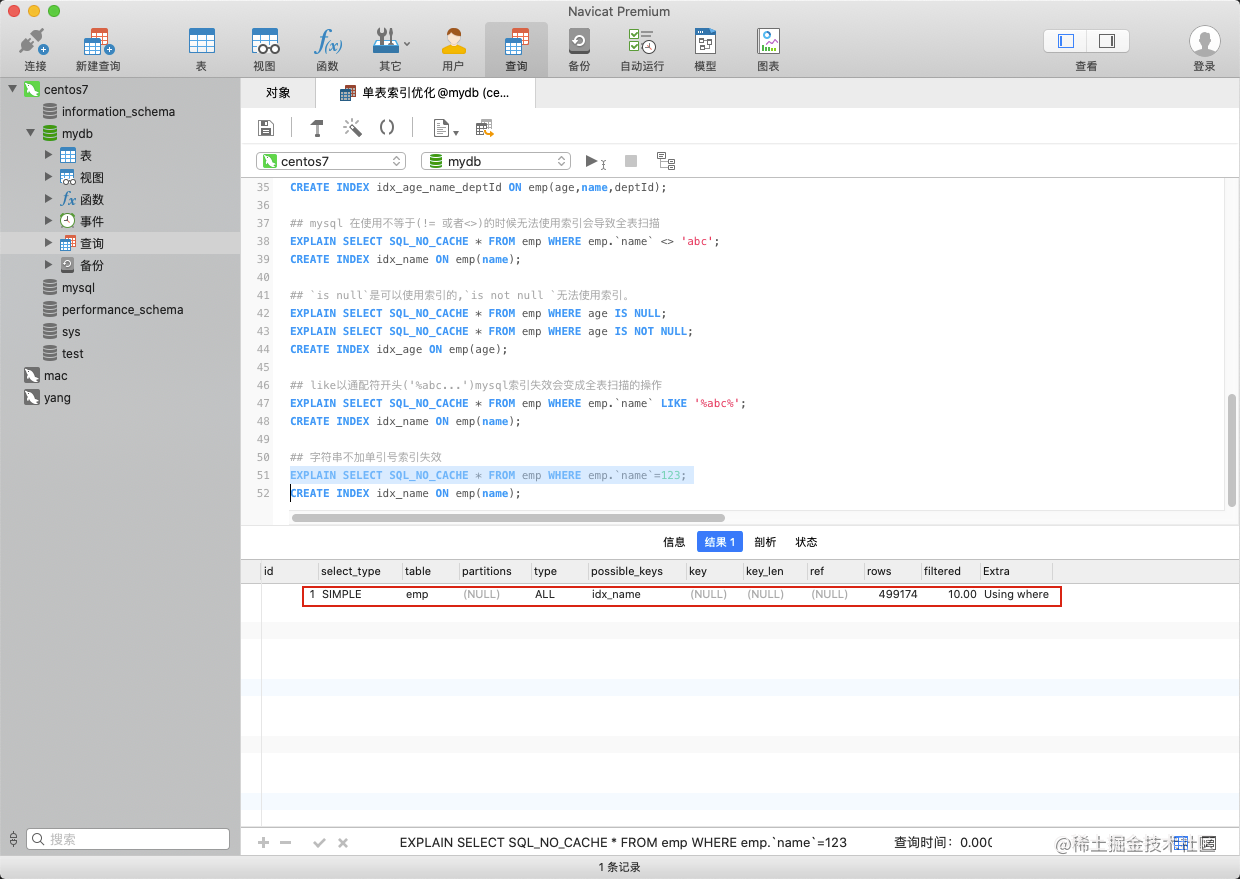``````## 字符串不加单引号索引失效
EXPLAIN SELECT SQL_NO_CACHE * FROM emp WHERE emp.`name`=123;
CREATE INDEX idx_name ON emp(name);

### 总结

1. 不在索引列上做任何操作（计算、函数、(自动or手动)类型转换），会导致索引失效而转向全表扫描。
2. 存储引擎不能使用索引中范围条件右边的列
3. mysql 在使用不等于(!= 或者<>)的时候无法使用索引会导致全表扫描
4. is not null 也无法使用索引,但是is null是可以使用索引的
5. like以通配符开头('%abc...')mysql索引失效会变成全表扫描的操作
6. 字符串不加单引号索引失效（出现自动类型转换）

Where语句索引是否被使用
where a = 3Y，使用到a
where a = 3 and b = 5Y,使用到a，b
where a = 3 and b = 5 and c = 4Y,使用到a,b,c
where b = 3 或者 where b = 3 and c = 4 或者 where c = 4N
where a = 3 and c = 5使用到a， 但是c不可以，b中间断了
where a = 3 and b > 4 and c = 5使用到a和b， c不能用在范围之后，b断了
where a is null and b is not nullis null 支持索引 但是is not null 不支持,所以 a 可以使用索引,但是 b不可以使用
where a <> 3不能使用索引
where abs(a) =3不能使用 索引
where a = 3 and b like 'kk%' and c = 4Y,使用到a,b,c
where a = 3 and b like '%kk' and c = 4Y,只用到a
where a = 3 and b like '%kk%' and c = 4Y,只用到a
where a = 3 and b like 'k%kk%' and c = 4Y,使用到a,b,c

1. 对于单键索引，尽量选择针对当前query过滤性更好的索引
2. 在选择组合索引的时候，当前Query中过滤性最好的字段在索引字段顺序中，位置越靠前越好。
3. 在选择组合索引的时候，尽量选择可以能够包含当前query中的where字句中更多字段的索引
4. 在选择组合索引的时候，如果某个字段可能出现范围查询时，尽量把这个字段放在索引次序的最后面
5. 书写sql语句时，尽量避免造成索引失效的情况

## 关联查询

``````CREATE TABLE IF NOT EXISTS `class` (
`id` INT(10) UNSIGNED NOT NULL AUTO_INCREMENT,
`card` INT(10) UNSIGNED NOT NULL,
PRIMARY KEY (`id`)
);
CREATE TABLE IF NOT EXISTS `book` (
`bookid` INT(10) UNSIGNED NOT NULL AUTO_INCREMENT,
`card` INT(10) UNSIGNED NOT NULL,
PRIMARY KEY (`bookid`)
);

INSERT INTO class(card) VALUES(FLOOR(1 + (RAND() * 20)));
INSERT INTO class(card) VALUES(FLOOR(1 + (RAND() * 20)));
INSERT INTO class(card) VALUES(FLOOR(1 + (RAND() * 20)));
INSERT INTO class(card) VALUES(FLOOR(1 + (RAND() * 20)));
INSERT INTO class(card) VALUES(FLOOR(1 + (RAND() * 20)));
INSERT INTO class(card) VALUES(FLOOR(1 + (RAND() * 20)));
INSERT INTO class(card) VALUES(FLOOR(1 + (RAND() * 20)));
INSERT INTO class(card) VALUES(FLOOR(1 + (RAND() * 20)));
INSERT INTO class(card) VALUES(FLOOR(1 + (RAND() * 20)));
INSERT INTO class(card) VALUES(FLOOR(1 + (RAND() * 20)));
INSERT INTO class(card) VALUES(FLOOR(1 + (RAND() * 20)));
INSERT INTO class(card) VALUES(FLOOR(1 + (RAND() * 20)));
INSERT INTO class(card) VALUES(FLOOR(1 + (RAND() * 20)));
INSERT INTO class(card) VALUES(FLOOR(1 + (RAND() * 20)));
INSERT INTO class(card) VALUES(FLOOR(1 + (RAND() * 20)));
INSERT INTO class(card) VALUES(FLOOR(1 + (RAND() * 20)));
INSERT INTO class(card) VALUES(FLOOR(1 + (RAND() * 20)));
INSERT INTO class(card) VALUES(FLOOR(1 + (RAND() * 20)));
INSERT INTO class(card) VALUES(FLOOR(1 + (RAND() * 20)));
INSERT INTO class(card) VALUES(FLOOR(1 + (RAND() * 20)));

INSERT INTO book(card) VALUES(FLOOR(1 + (RAND() * 20)));
INSERT INTO book(card) VALUES(FLOOR(1 + (RAND() * 20)));
INSERT INTO book(card) VALUES(FLOOR(1 + (RAND() * 20)));
INSERT INTO book(card) VALUES(FLOOR(1 + (RAND() * 20)));
INSERT INTO book(card) VALUES(FLOOR(1 + (RAND() * 20)));
INSERT INTO book(card) VALUES(FLOOR(1 + (RAND() * 20)));
INSERT INTO book(card) VALUES(FLOOR(1 + (RAND() * 20)));
INSERT INTO book(card) VALUES(FLOOR(1 + (RAND() * 20)));
INSERT INTO book(card) VALUES(FLOOR(1 + (RAND() * 20)));
INSERT INTO book(card) VALUES(FLOOR(1 + (RAND() * 20)));
INSERT INTO book(card) VALUES(FLOOR(1 + (RAND() * 20)));
INSERT INTO book(card) VALUES(FLOOR(1 + (RAND() * 20)));
INSERT INTO book(card) VALUES(FLOOR(1 + (RAND() * 20)));
INSERT INTO book(card) VALUES(FLOOR(1 + (RAND() * 20)));
INSERT INTO book(card) VALUES(FLOOR(1 + (RAND() * 20)));
INSERT INTO book(card) VALUES(FLOOR(1 + (RAND() * 20)));
INSERT INTO book(card) VALUES(FLOOR(1 + (RAND() * 20)));
INSERT INTO book(card) VALUES(FLOOR(1 + (RAND() * 20)));
INSERT INTO book(card) VALUES(FLOOR(1 + (RAND() * 20)));
INSERT INTO book(card) VALUES(FLOOR(1 + (RAND() * 20)));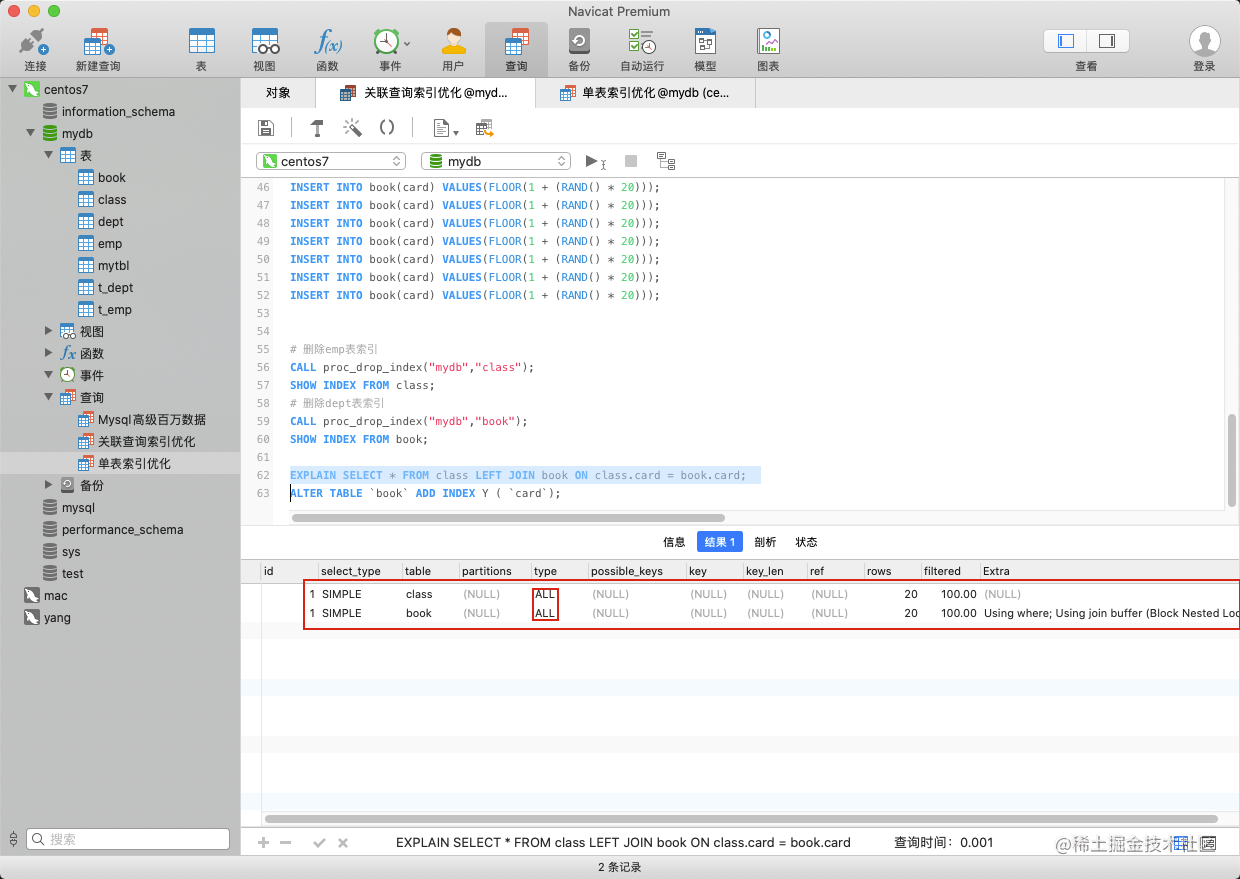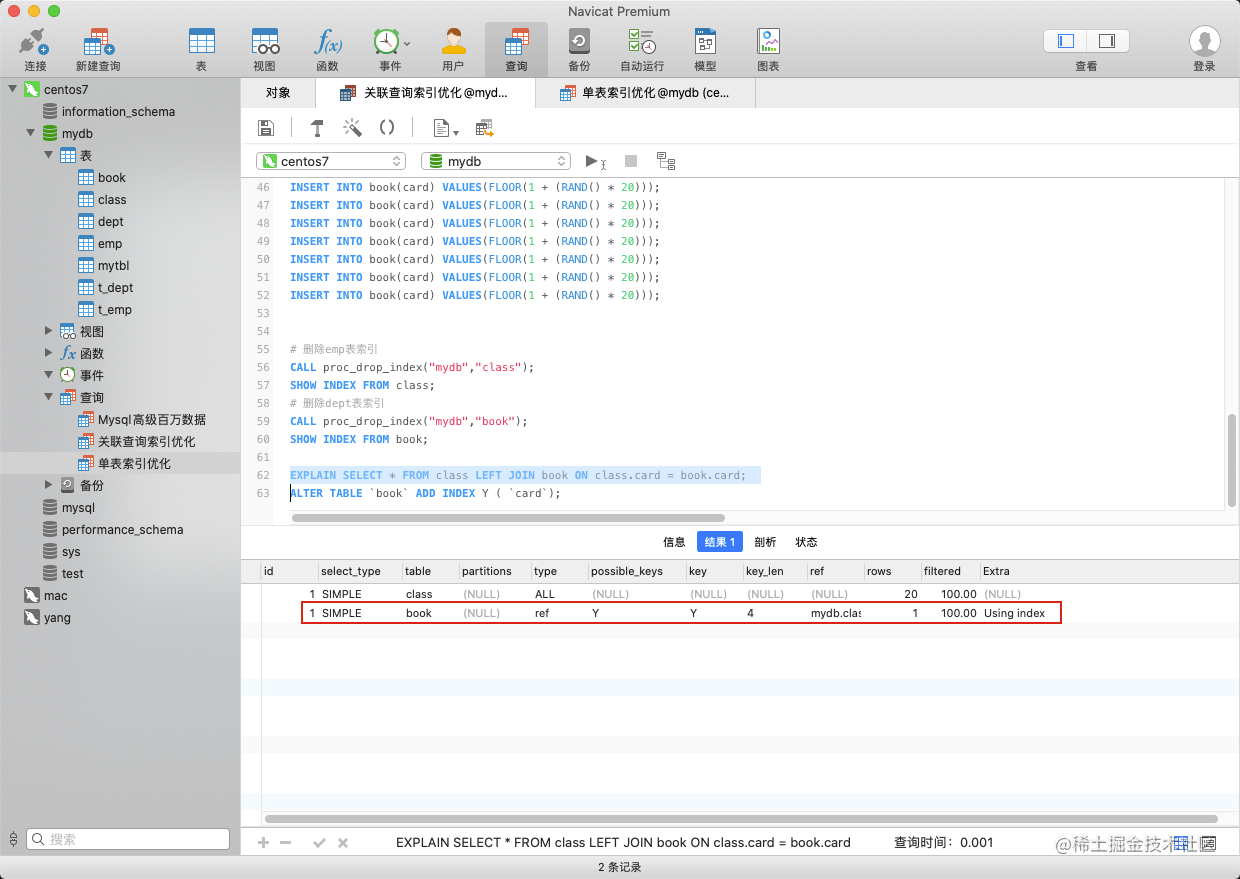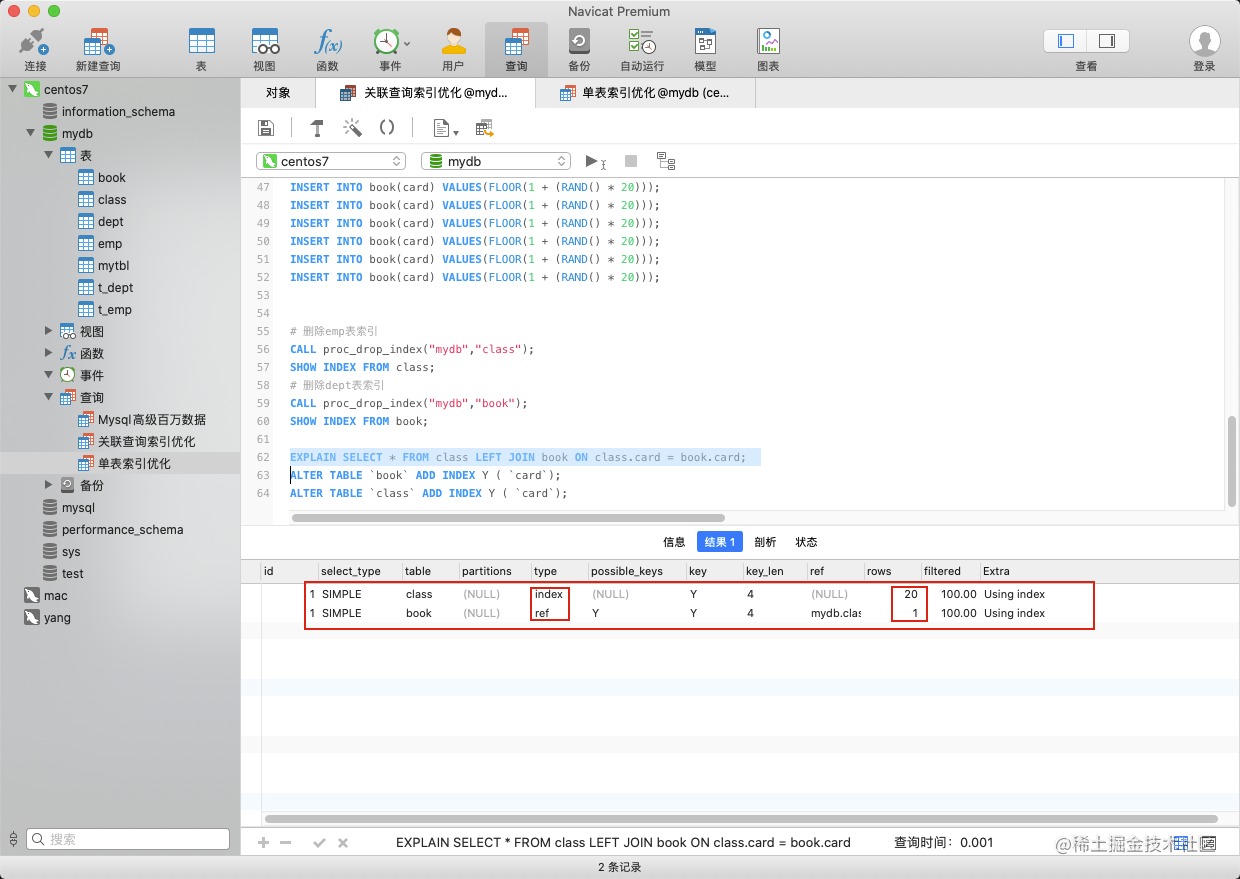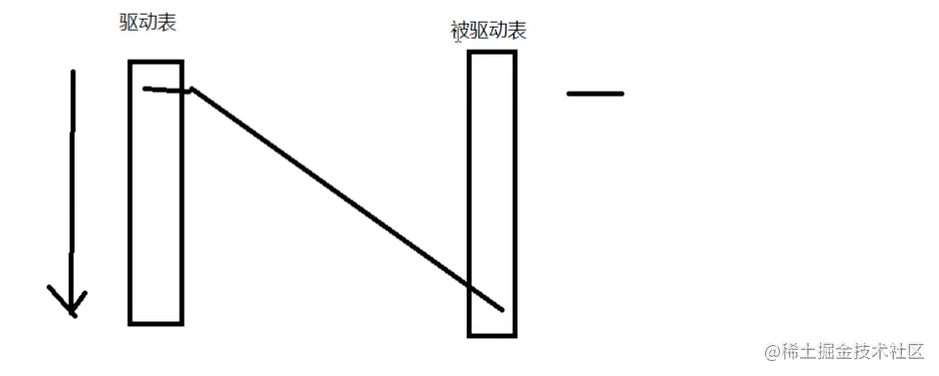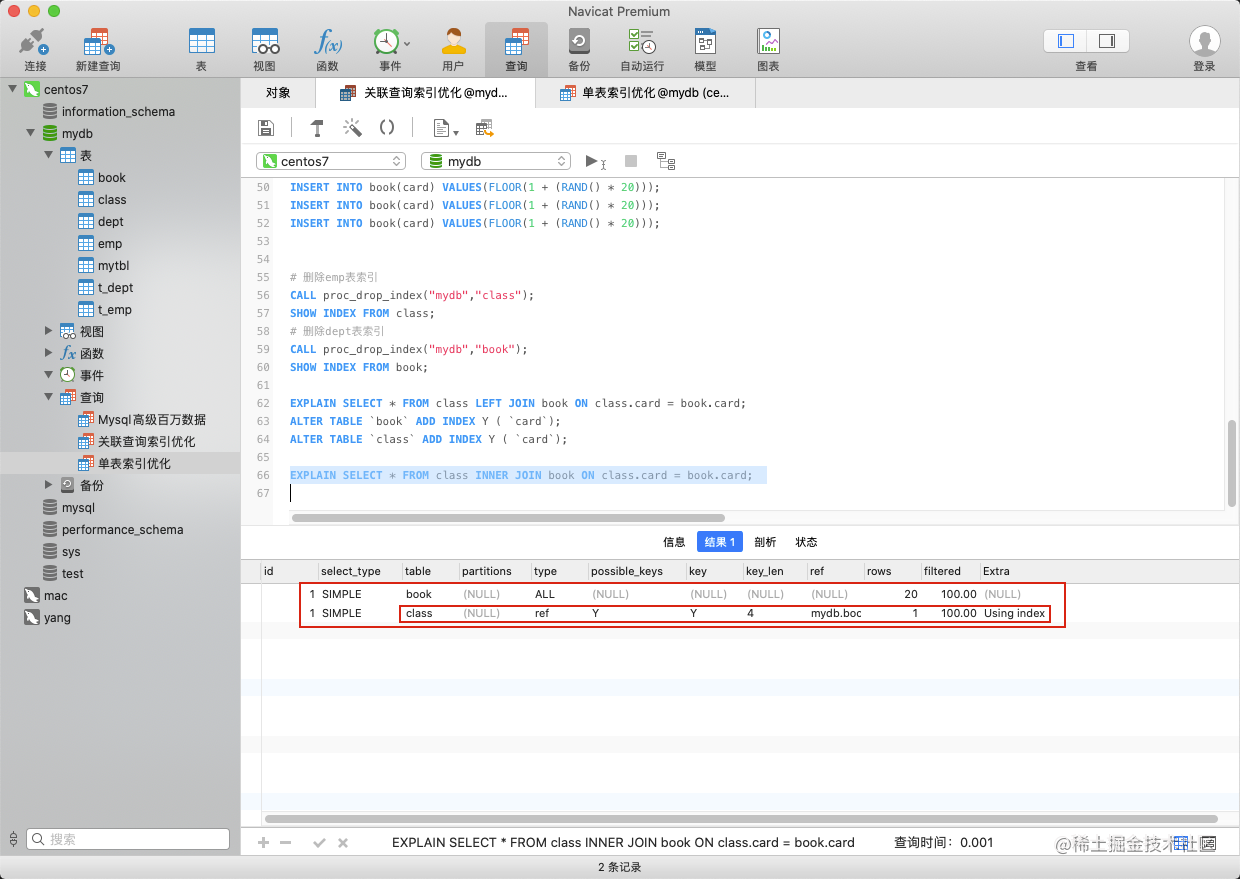id相同，按照由上至下的顺序执行，所以`book` 变成了驱动表，`class` 表变成了被驱动表。class的索引优化生效。

1. 保证被驱动表的join字段已经被索引
2. left join 时，选择小表作为驱动表，大表作为被驱动表。
3. inner join 时，mysql会自己帮你把小结果集的表选为驱动表。
4. 虚拟表无法建立索引
5. 子查询尽量不要放在被驱动表，有可能使用不到索引。
6. 能够直接多表关联的尽量直接关联，不用子查询。

## 子查询优化

``````SELECT * FROM t_emp a WHERE a.id NOT IN
(SELECT b.ceo FROM t_dept b where b.ceo IS NO NULL);

SELECT * FROM t_emp a LEFT JOIN t_dept b ON a.id = b.ceo
WHERE b.id IS NULL;

## 排序分组优化

### order by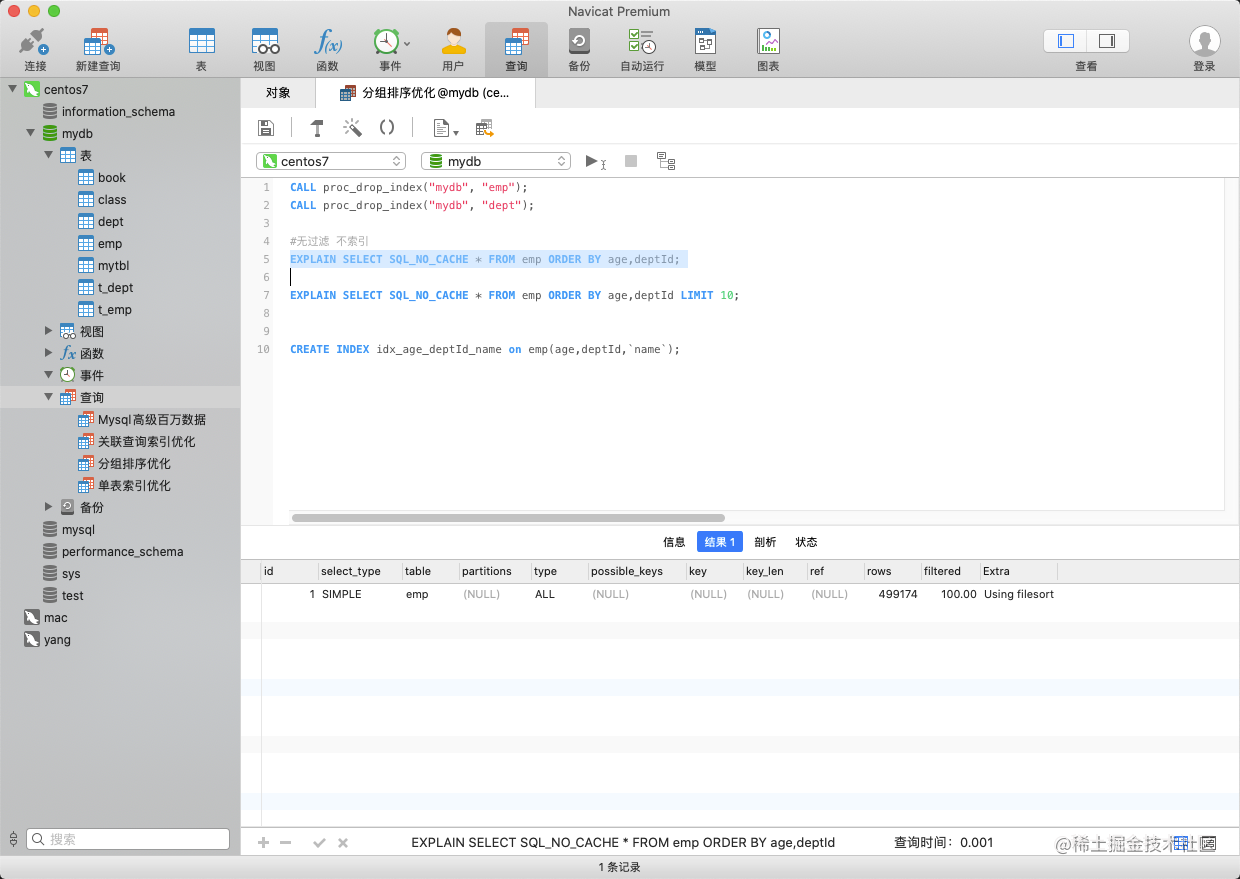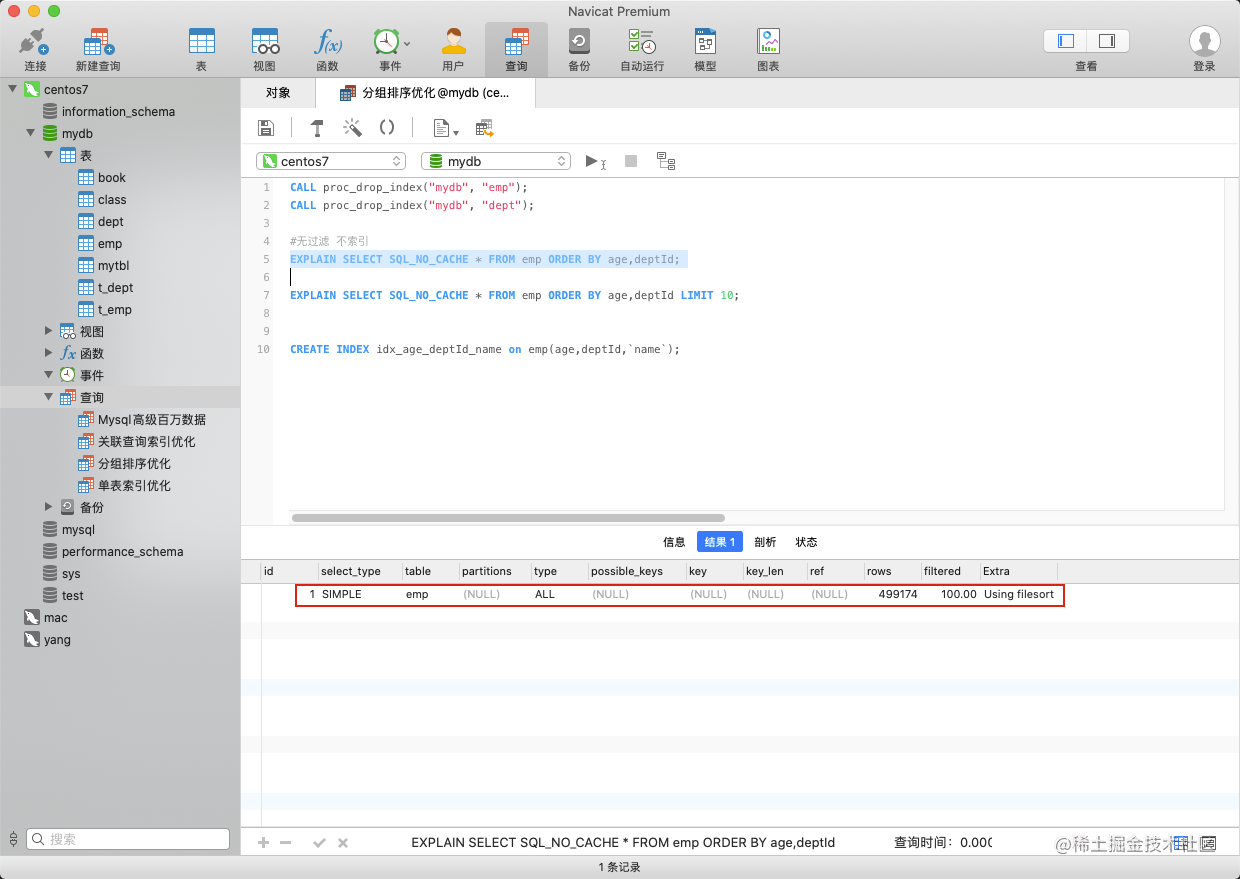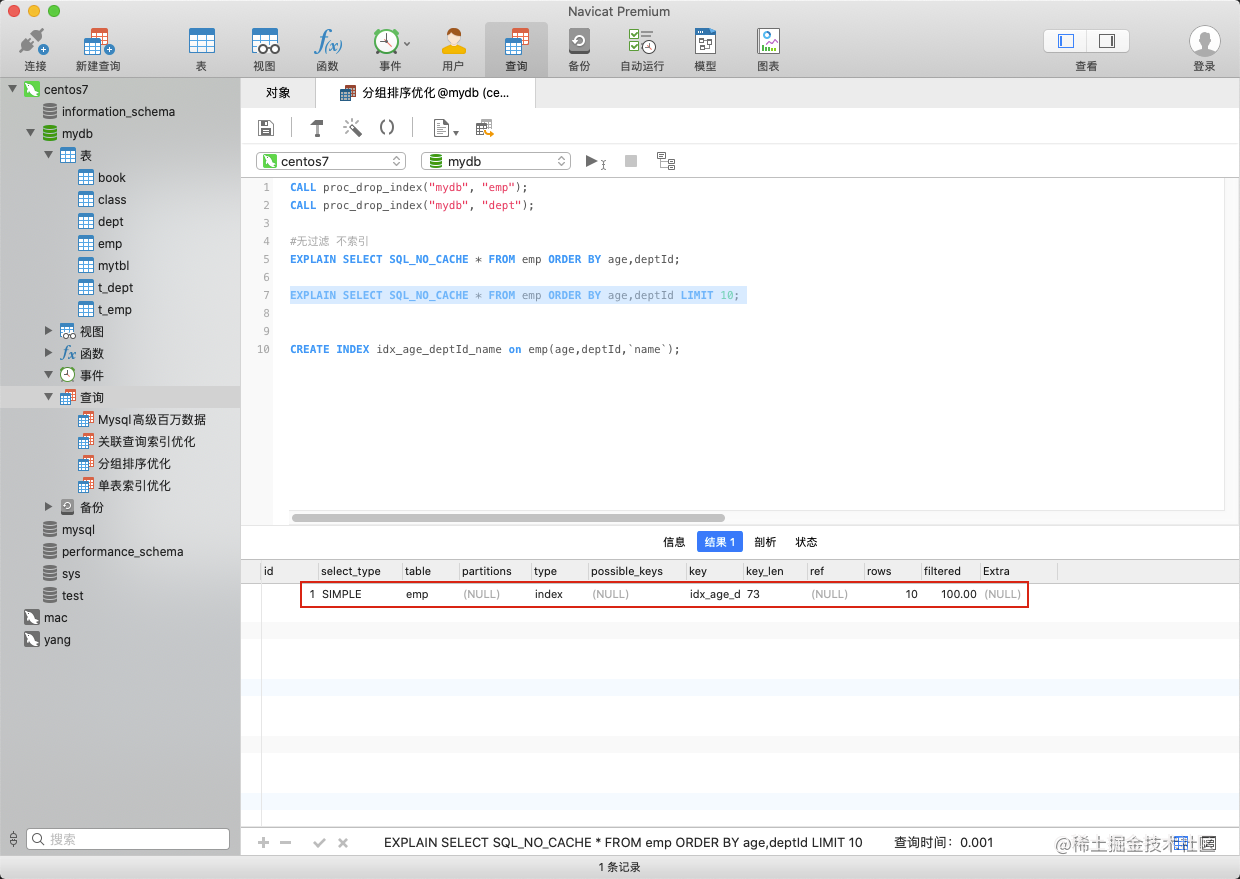``````#无过滤 不索引
EXPLAIN SELECT SQL_NO_CACHE * FROM emp ORDER BY age,deptId;

EXPLAIN SELECT SQL_NO_CACHE * FROM emp ORDER BY age,deptId LIMIT 10;

CREATE INDEX idx_age_deptId_name on emp(age,deptId,`name`);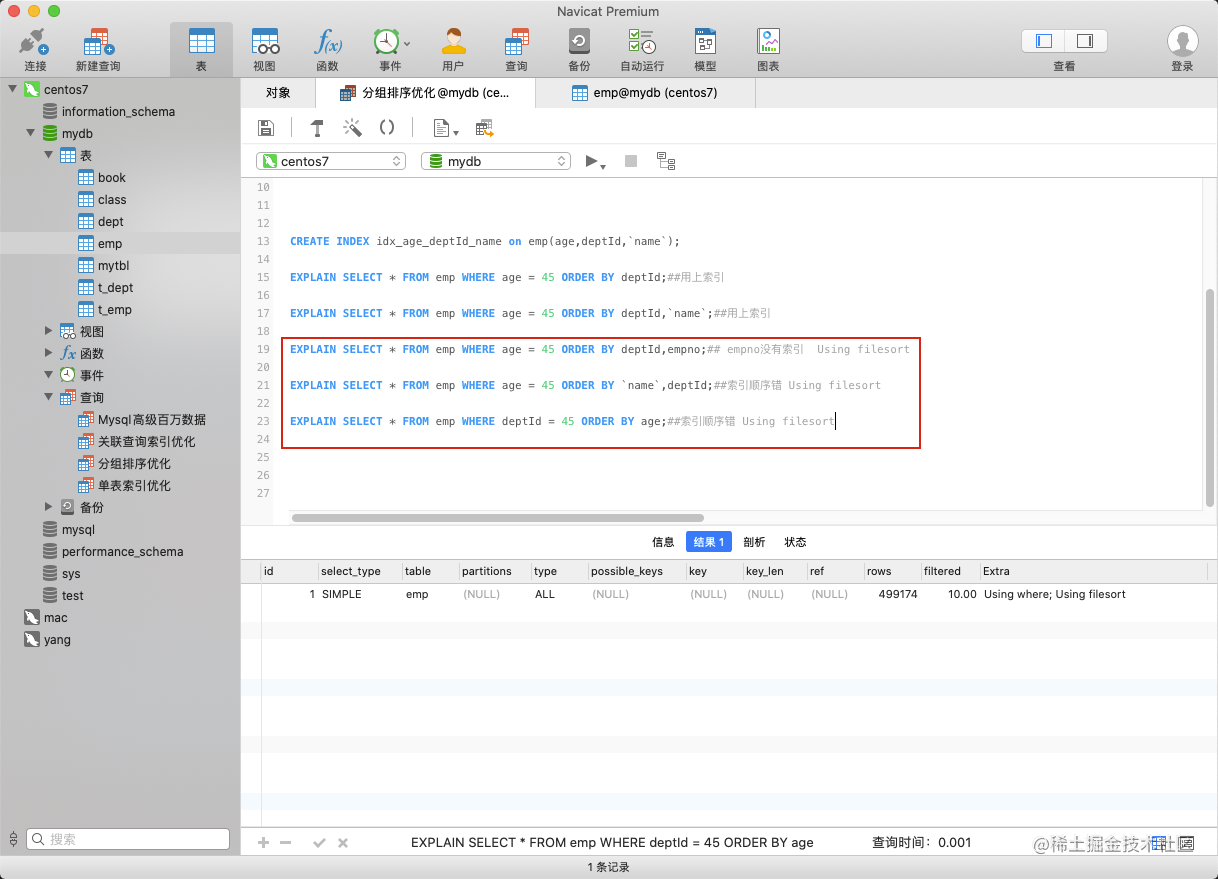``````CREATE INDEX idx_age_deptId_name on emp(age,deptId,`name`);

EXPLAIN SELECT * FROM emp WHERE age = 45 ORDER BY deptId;##用上索引

EXPLAIN SELECT * FROM emp WHERE age = 45 ORDER BY deptId,`name`;##用上索引

EXPLAIN SELECT * FROM emp WHERE age = 45 ORDER BY deptId,empno;## empno没有索引  Using filesort

EXPLAIN SELECT * FROM emp WHERE age = 45 ORDER BY `name`,deptId;##索引顺序错 Using filesort

EXPLAIN SELECT * FROM emp WHERE deptId = 45 ORDER BY age;##索引顺序错 Using filesort

where条件进行过滤，索引顺序要保证，不能错，不能断。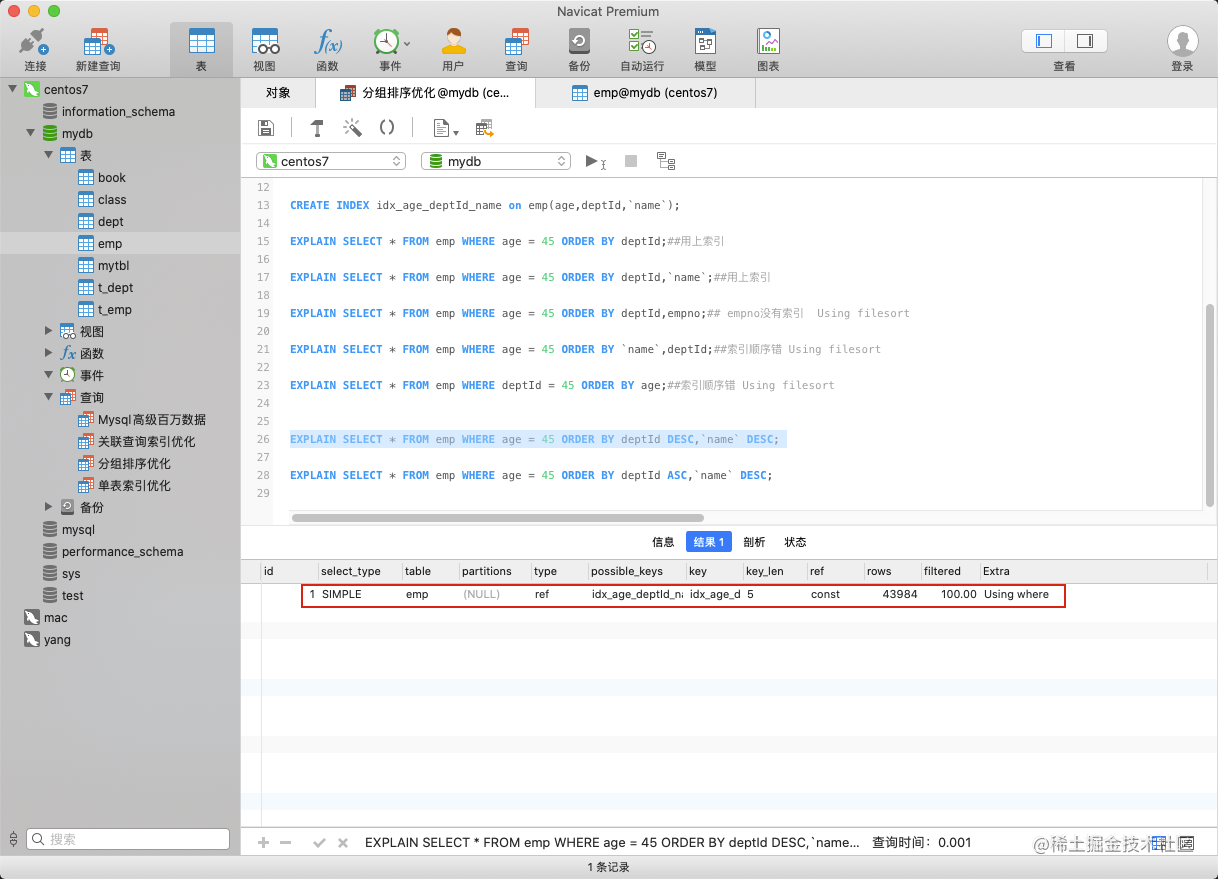### 索引选择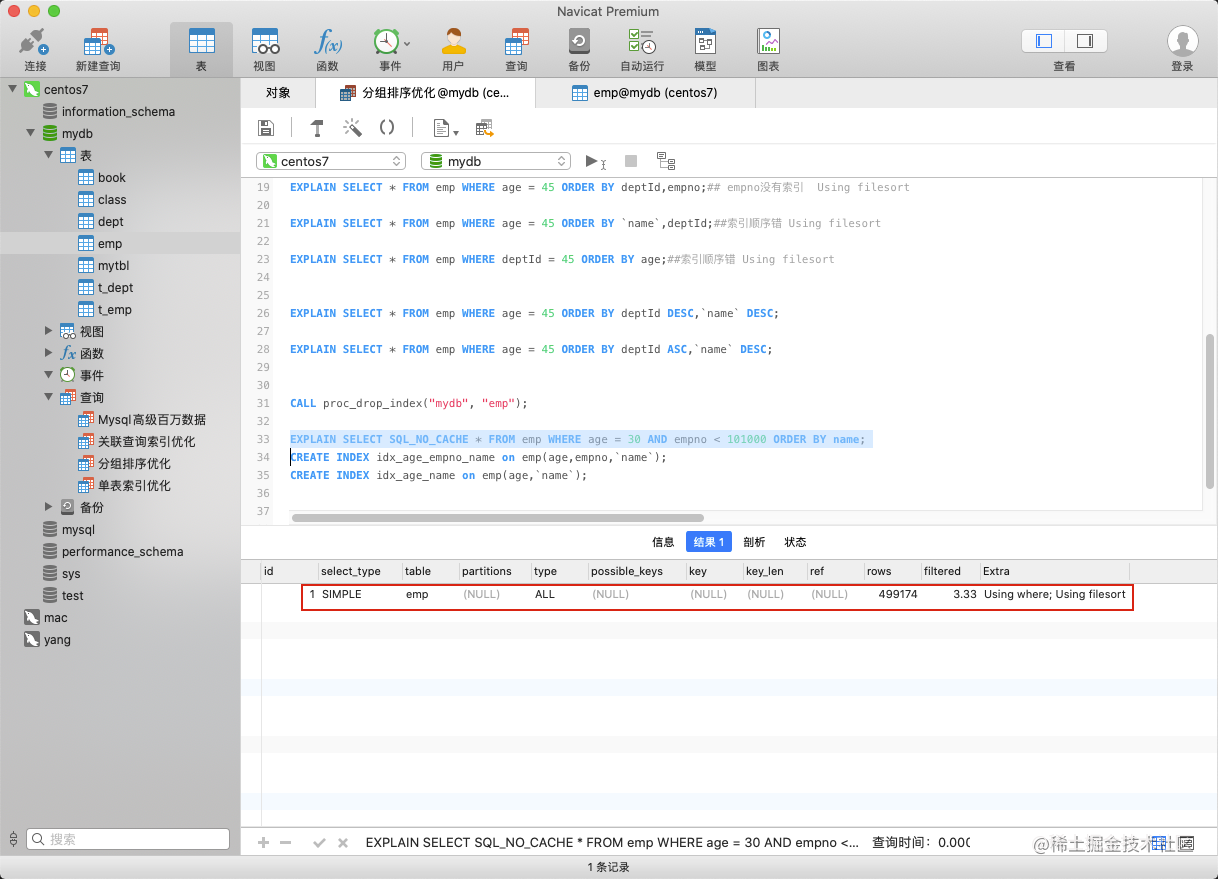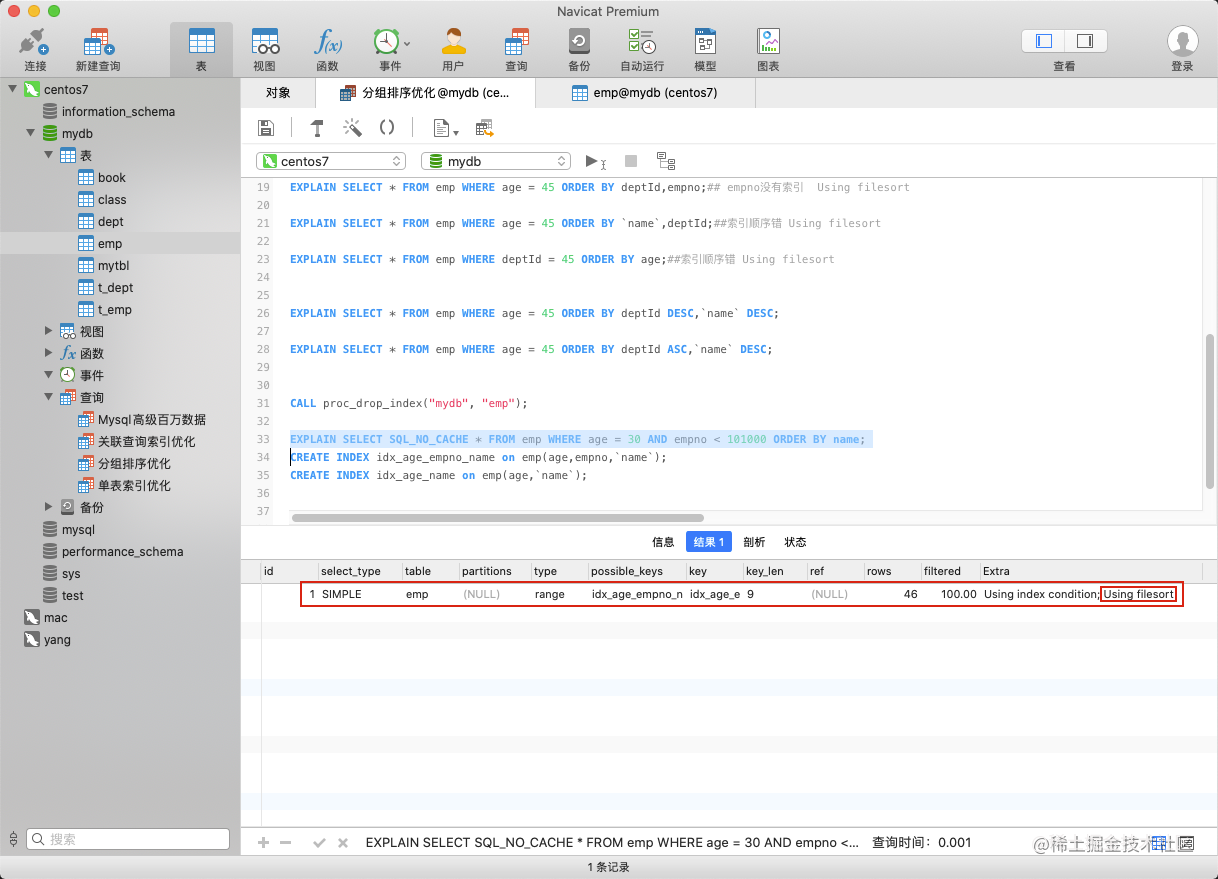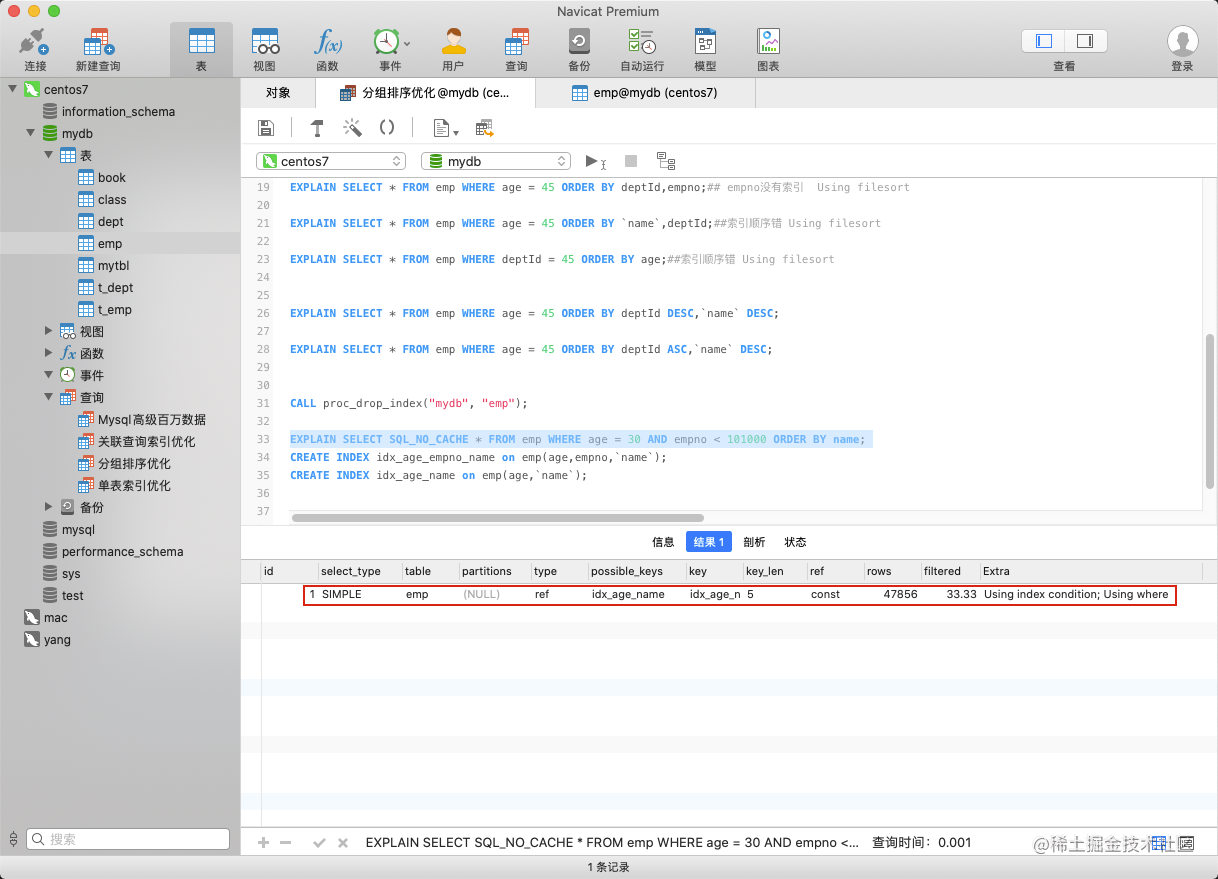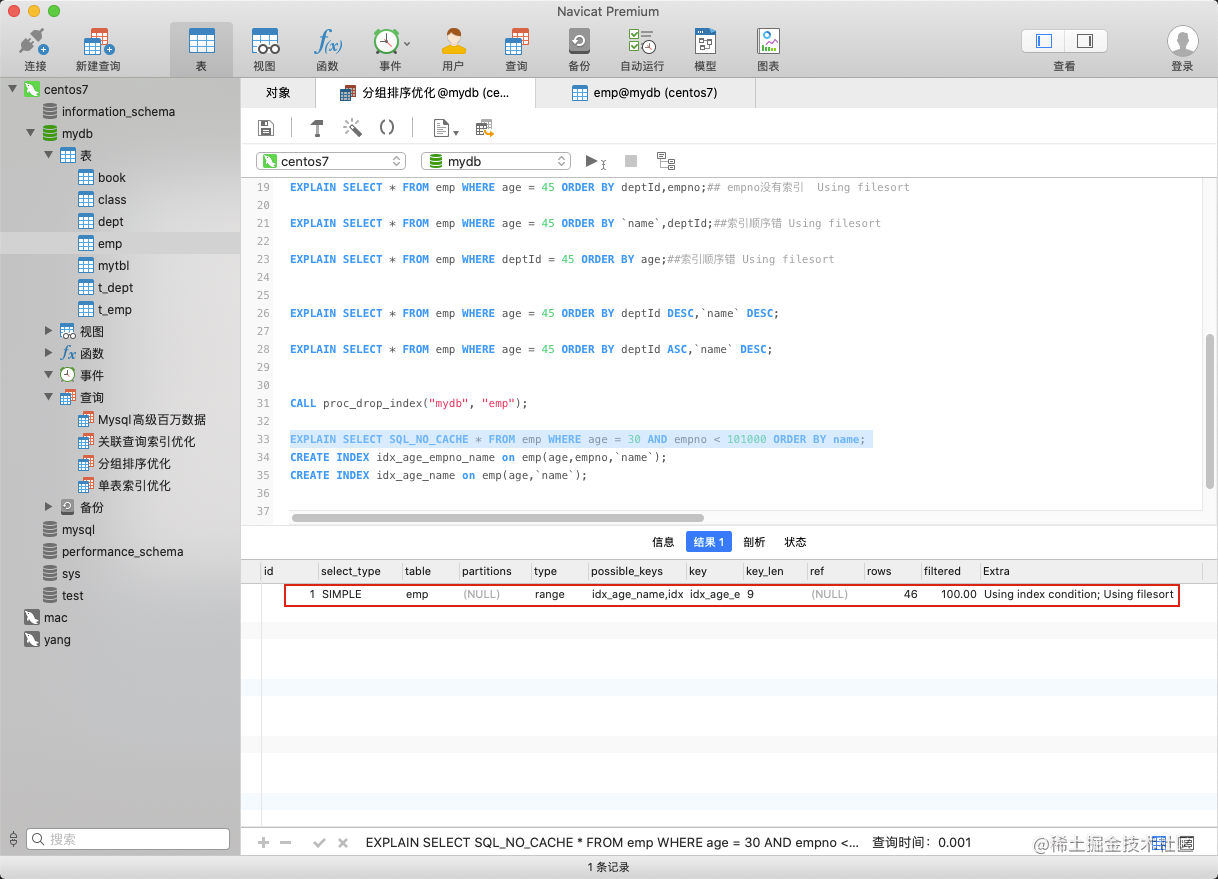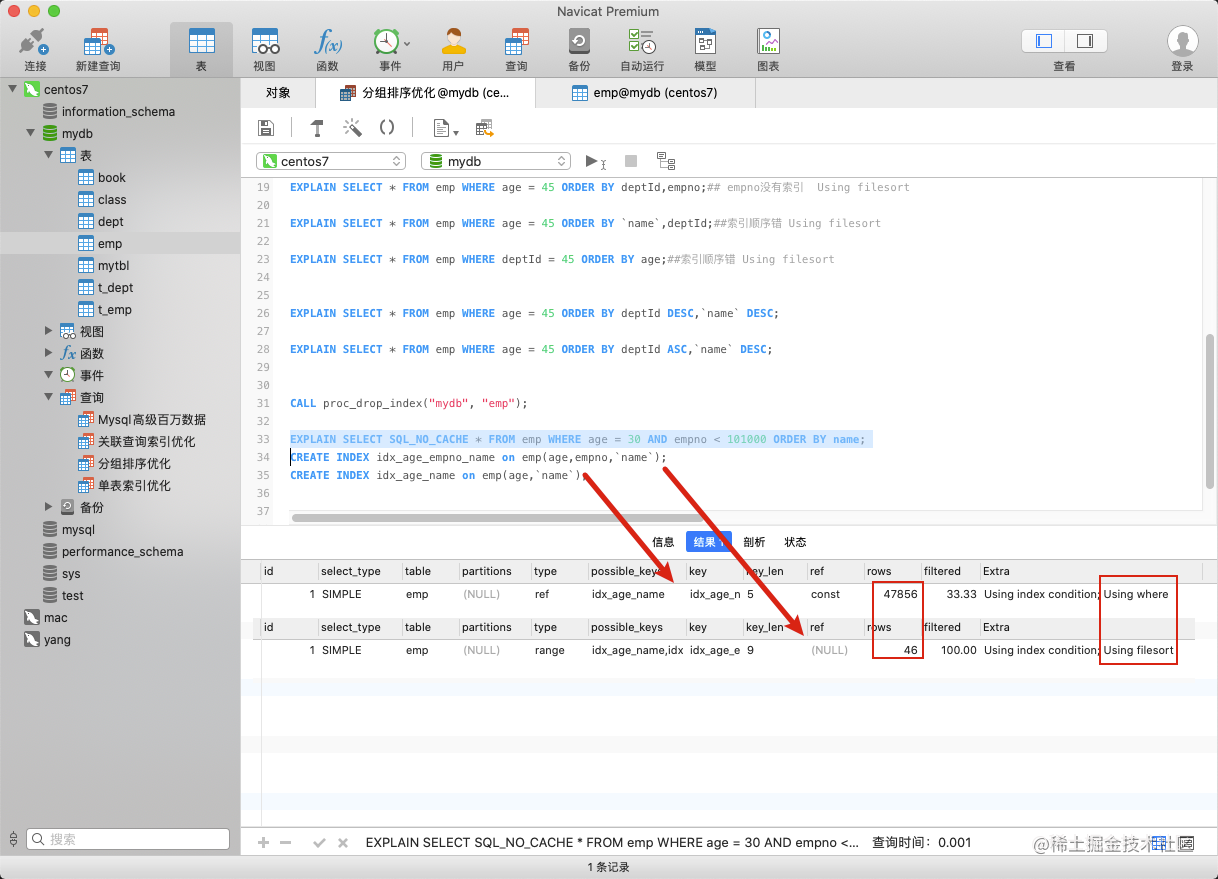### Using filesort

• 增大sort_buffer_size参数的设置
• 增大max_length_for_sort_data参数的设置
• 减少select 后面的查询的字段。

1. Order by时select * 是一个大忌只Query需要的字段， 这点非常重要。在这里的影响是：

• 当Query的字段大小总和小于max_length_for_sort_data 而且排序字段不是 TEXT|BLOB 类型时，会用改进后的算法——单路排序， 否则用老算法——多路排序。

• 两种算法的数据都有可能超出sort_buffer的容量，超出之后，会创建tmp文件进行合并排序，导致多次I/O，但是用单路排序算法的风险会更大一些,所以要提高sort_buffer_size。

2. 尝试提高 sort_buffer_size 不管用哪种算法，提高这个参数都会提高效率，当然，要根据系统的能力去提高，因为这个参数是针对每个进程的 1M-8M之间调整

3. 尝试提高 max_length_for_sort_data 提高这个参数， 会增加用改进算法的概率。但是如果设的太高，数据总容量超出sort_buffer_size的概率就增大，明显症状是高的磁盘I/O活动和低的处理器使用率. 1024-8192之间调整

### group by

group by 使用索引的原则几乎跟order by一致 ，唯一区别是groupby 即使没有过滤条件用到索引，也可以直接使用索引。

## 覆盖索引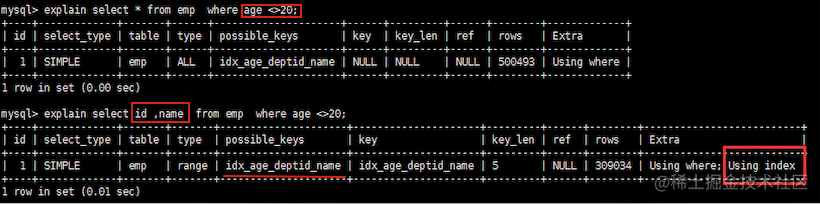`explain select * from emp where name like '%abc';`